Impact Factor 4.506 | CiteScore 4.5
More on impact ›

# Frontiers in Synaptic Neuroscience

## ORIGINAL RESEARCH article

Front. Synaptic Neurosci., 03 September 2010 | https://doi.org/10.3389/fnsyn.2010.00033

# Rate and pulse based plasticity governed by local synaptic state variables

• Endowed Chair of Highly Parallel VLSI Systems and Neural Microelectronics, Institute of Circuits and Systems, Faculty of Electrical Engineering and Information Science, University of Technology Dresden, Dresden, Sachsen, Germany

Classically, action-potential-based learning paradigms such as the Bienenstock–Cooper–Munroe (BCM) rule for pulse rates or spike timing-dependent plasticity for pulse pairings have been experimentally demonstrated to evoke long-lasting synaptic weight changes (i.e., plasticity). However, several recent experiments have shown that plasticity also depends on the local dynamics at the synapse, such as membrane voltage, Calcium time course and level, or dendritic spikes. In this paper, we introduce a formulation of the BCM rule which is based on the instantaneous postsynaptic membrane potential as well as the transmission profile of the presynaptic spike. While this rule incorporates only simple local voltage- and current dynamics and is thus neither directly rate nor timing based, it can replicate a range of experiments, such as various rate and spike pairing protocols, combinations of the two, as well as voltage-dependent plasticity. A detailed comparison of current plasticity models with respect to this range of experiments also demonstrates the efficacy of the new plasticity rule. All experiments can be replicated with a limited set of parameters, avoiding the overfitting problem of more involved plasticity rules.

## Introduction

One of the major research areas of neurobiology is long-term learning (i.e., plasticity) of synapses in neural tissue (Koch, 1999; Lisman and Spruston, 2005; Pfister and Gerstner, 2006; Morrison et al., 2008). Synapses are the contact points between neurons, where information from the sending neuron arrives at the so-called presynaptic side and is transmitted via the synapse as a postsynaptic current (PSC) pulse to the receiving neuron. The concept of plasticity is used to describe the phenomenon that certain types of pre- and postsynaptic stimuli can have long-lasting effects on the efficacy of this transmission (Bienenstock et al., 1982; Dudek and Bear, 1992; Morrison et al., 2008), i.e., the size of the PSC, ranging from days up to a year. These phenomena are called long-term depression (LTD) for diminished synaptic responses and long-term potentiation (LTP) for enhanced responses. Depending on the induction protocol, spike rates (Dudek and Bear, 1992; Mayford et al., 1995; Wang and Wagner, 1999; Abraham et al., 2001) or different spike patterns (Markram et al., 1997; Bi and Poo, 1998; Sjöström et al., 2001; Froemke and Dan, 2002) have been found to elicit changes in synaptic weight. Various models have tried to incorporate the principal experimental findings, e.g., in implementations of the classical rate-based Bienenstock–Cooper–Munroe (BCM) rule (Bienenstock et al., 1982; Shouval et al., 2002; Kurashige and Sakai, 2006) or the newer spike timing-dependent plasticity (STDP) rule (Badoual et al., 2006; Morrison et al., 2008). Since both rules describe phenomena which have been shown to coexist at the same synapse, several models try to achieve a synthesis of both rules (Senn, 2002; Izhikevich and Desai, 2003; Pfister and Gerstner, 2006; Benuskova and Abraham, 2007).

However, these models usually represent only a statistical average of synaptic responses, as the relevant experiments exhibit a large spread in experimental data (Dudek and Bear, 1992; Bi and Poo, 1998; Froemke and Dan, 2002). This statistical spread may be explained by the fact that relevant local plasticity variables are only unreliably influenced by action potentials (Lisman and Spruston, 2005). Consequently, some experiments seem to indicate that LTP and LTD can be more reliably induced qualitatively as well as quantitatively (i.e., with less variability in the direction and amount of weight change) if local variables are directly influenced, e.g., by evoking dendritic spikes (Holthoff et al., 2006) or by setting the membrane potential artificially (Artola et al., 1990; Ngezahayo et al., 2000; Sjöström et al., 2001). Thus, we hypothesize that the governing variables of plasticity are based on the local environment of the synapse such as the local depolarization or Ca2+ levels (Aihara et al., 2007).

Motivated by these findings, several models have tried to incorporate a dependence of plasticity on the Ca2+ concentration or the membrane potential. However, most of the Ca2+ based models, while able to reproduce BCM-type plasticity, result in unrealistic STDP curves (Shouval et al., 2002; Kurashige and Sakai, 2006; Shah et al., 2006). In addition, the reproduction of higher-order spike interaction effects such as spike triplets (Froemke and Dan, 2002) are either not attempted by the authors or produce results only partially consistent with experimental data (Shah et al., 2006). As well, due to their biophysical motivation, these models tend to be very complex and thus computationally expensive and difficult to analyze mathematically.

Models incorporating the membrane potential have mostly been influenced by computational aspects (Saudargiene et al., 2004; Toyoizumi et al., 2005; Pfister et al., 2006; Baras and Meir, 2007). Pfister et al. (2006) and Saudargiene et al. (2004) have tried to link the learning capability of a neuron to its inherent temporal dynamics and to its structure. STDP-type plasticity is derived for both models, while BCM behavior is not explicitly shown. In the context of reward based learning (Baras and Meir, 2007) and optimal information transmission (Toyoizumi et al., 2005), two further authors show BCM-like behavior based on the timing of pre- and postsynaptic spikes and the membrane potential, but their actual weight change curve in STDP terms is unclear. In general, as the above authors were mainly interested in computational aspects, there was no effort to show the generalization capability of their models by explicitly reproducing experimental protocols. As in the case of the Ca2+ based plasticity, the model description is on average very complex, with multiple different time traces/equations interacting with each other. One notable exception is the model described in Clopath et al. (2008a, 2010), where an STDP rule is extended by voltage thresholds and a dependence on the membrane potential to reproduce several experimental findings in the areas of rate-, pulse-, and voltage-based plasticity.

As can be seen from the above, there are various models which can reproduce/explain individual facets of the experimental data, and also some more general models linking two of the three major groups of plasticity phenomena (i.e., voltage, rate, spike timing), but there is only a very limited number of efforts which would link all three.

In the following, we will fully develop a model introduced in an earlier paper (Partzsch et al., 2008) which tries to achieve such a synthesis. In contrast to the extended STDP of Clopath et al. (2010), we start out with the original formulation of the BCM rule, but interpret its state variables for pre- and postsynaptic activity as synaptic current respectively membrane potential and its threshold as membrane resting potential, arriving at a plasticity dependent on local synaptical dynamics, which we call local correlation plasticity (LCP). In Section “Plasticity Model Review,” we list a number of experimental benchmarks, followed by a classification scheme for current plasticity models. Based on this framework, we give an overview of plasticity models (see Model Comparison), which serves as a database against which we can compare our models. In Section “Local Correlation Plasticity,” we introduce the LCP variant of Partzsch et al. (2008), which uses a simple spike response model (SRM) for its postsynaptic dynamics. In Section “Neuron Dynamics: Leaky Integrate-and-Fire” we introduce a leaky integrate-and-fire (LIAF) neuron which augments LCP with more realistic postsynaptic dynamics. Following, we relate the (biophysical) parameters of the SRM neuron to the (phenomenological) parameters from STDP experiments (see Analysis Results). Also, we show the relationship between the sliding threshold of the original BCM and the parameters inherent in our LCP rule. In Section “Simulation Results,” we give simulation results for various kinds of experimental stimulation protocols, subsequently discussing the limits of LCP using the SRM neuron model as evident from the simulations. Section “LCP with LIAF Neuron” shows results on how LCP with the LIAF neuron model resolves the discrepancies of the previous section. Also, the extension enables our model to reproduce several more experimental results. Finally, repercussions and limits of our model as well as further research directions such as the incorporation of a more realistic neuron model are discussed in Section “Discussion.”

## Materials and Methods

### Plasticity Model Review

#### Benchmark experiments

In order to enable a qualified comparison of the LCP rule with current state-of-the-art, we have gathered a listing of BCM- and STDP-related biological experiments, describing different facets of LTP and LTD induction. The experiment summary in Table 1 includes the pulse induction protocol necessary for replicating the experiment with a plasticity model, as well as the experimental conditions as derived from the papers or related literature. The experimental conditions are mainly given to judge how similar the setup is for different protocols, i.e., should a plasticity model be able to replicate several experiments using the same parameter set, or is a change in model parameters justified by the change in experimental setup. For example, differences in the age of the experimental animal significantly affect plasticity (Disterhoft and Oh, 2006; Lante et al., 2006), which justifies using different parameter sets, while differences in preparation (i.e., slice or dissociated) may or may not change neuron behavior (Du and Bradley, 1996; Taubenfeld et al., 2002).

Table 1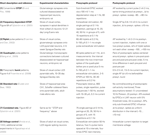Table 1. Listing of plasticity experiments, the numbers and keywords assigned in bold font to the single experiments are used for later reference in the text.

Starting point for the above listing is conventional STDP (Bi and Poo, 1998), i.e., the plasticity or change in PSC amplitude evoked by several repetitions of single presynaptic-postsynaptic spike pairings (at various positive and negative time differences) at a low repetition frequency. In related experiments, Sjöström et al. (2001) have also tested the frequency dependence of plasticity for pre-post and post-pre pairings. They found an increasing potentiation effect in both cases, leading to a transition from overall depression to potentiation in the post-pre case at approx. 30 Hz (cf. Figure 10). Froemke and Dan (2002) have shown that spike pairings do not sum linearly, for example that spike triplets in post-pre-post order cannot be treated as separate pairings post-pre and pre-post when computing the cumulative weight change. Wang et al. (2005) also carried out triplet experiments, which show somewhat differing behavior. The major difference seems to occur for post-pre-post triplets with low time differences, where Wang et al. (2005) observe postsynaptic facilitation for the second postsynaptic pulse, resulting in amplified LTP, contrary to the postsynaptic depression seen in Froemke and Dan (2002) for the same triplets (cf. circles in the lower right quadrant of Figures 7A,B). This difference between the enhancing and depressing effect for these particular triplets seems to be fundamental, i.e., models which accommodate one effect cannot replicate the other. Thus, we classify a model as compatible with experimental triplet results if it can emulate one of the two results. A further common spiking protocol is the quadruplet protocol, consisting of a post-pre and a pre-post pair with equal time difference Δt that are separated by a short time interval T. Wang et al. (2005) have performed a series of such experiments, using Δt = 5 ms. Subsets of the above protocols (conventional STDP, STDP and rate, triplets, and quadruplets) are commonly used in the verification of newly introduced plasticity rules (Froemke and Dan, 2002; Senn, 2002; Pfister and Gerstner, 2006; Shah et al., 2006).

The experiments in Froemke et al. (2006) examine plasticity with respect to bursts and individual spike timing. The experiment documented in Figure 2 of Froemke et al. (2006) uses short bursts of 5 spikes at different burst frequencies and individual pre-post-spike timing of 6 ms. This is very similar to the experiment in Figure 8A in Sjöström et al. (2001), (frequency-dependent STDP), so we did not include this experiment in our table. We also did not include the experiment of Figure 3 in Froemke et al. (2006), since weight saturation or dependence on initial weight is a very active topic and few assured facts exist (Kepecs et al., 2002; Standage and Trappenberg, 2007; Zou and Destexhe, 2007). We did include the post-pre-burst and pre-burst-post pairing protocol of Figure 4 in Froemke et al. (2006) as a testcase, since this protocol can very likely not be replicated even with models that take triplet effects into account (Froemke et al., 2006). Figures 6A,B of Froemke et al. (2006) show that there is no significant difference between conventional post-pre-post triplets and post-burst-pre-post, i.e., triplet models should be able to express this behavior, therefore we also did not include this protocol. The crossover from LTD to LTP for the post-pre-post-burst stimulation protocol of Figures 6C,D, although not replicated by the model of Froemke and Dan (2002), can be reproduced by conventional nearest-neighbor STDP and also by LCP with SRM (see Figure 9). Since this protocol seems not to represent a major challenge to plasticity models, we also did not include it.

The behavior of synapses with respect to pulse rates is usually characterized by the BCM formulation (Bienenstock et al., 1982). That is, a low postsynaptic rate produces LTD, with an increase in frequency corresponding to a gradual reduction of LTD. Above a certain threshold, LTP is produced, which increases with postsynaptic rate. The presynaptic rate scales this behavior. The postsynaptic frequency threshold separating LTD and LTP is variable, i.e., it changes its value dependent on past activity at the synapse (Wang and Wagner, 1999).

A synthetic version of a rate protocol is usually employed for confirming compatibility with the BCM paradigm (Senn, 2002; Izhikevich and Desai, 2003; Toyoizumi et al., 2005; Baras and Meir, 2007), neglecting a detailed replication of experimental BCM-type results, although some authors employ more realistic stimulation protocols for their models (Shouval et al., 2002; Benuskova and Abraham, 2007). In contrast to that, two experimental rate protocols are included in Table 1 to ensure accuracy of the LCP rule with respect to actual experiments. The first pulse rate experiment uses a presynaptically applied high frequency tetanus, while the postsynaptic side seems not to have been controlled (Dudek and Bear, 1992). Since there is no data about the postsynaptic side, we use three different settings to cover a wide range of possible postsynaptic activity: the first assumption is that of a silent, non-spiking slice preparation, i.e., neither the presynaptic tetanus nor background activity does result in firing of the neuron, the membrane potential is only depolarized in the sub-threshold regime due to the presynaptic currents. The second setting assumes that the firing of the neuron is completely driven by unspecific background activity, modeled by a 10 Hz Poisson process. In contrast, the third setting neglects the background activity and assumes that the presynaptic tetanus drives the postsynaptic firing, modeled by an LIAF neuron with threshold noise (Gerstner and Kistler, 2002), which is described in detail in Section “Neuron Dynamics: Leaky Integrate-and-Fire.” This assumption results in strongly correlated pre- and postsynaptic firing, similar to the postsynaptic reconstructions of Beggs (2001) and Standage et al. (2007). The first and third assumptions are also very similar to the postsynaptic reconstruction of experiment (6) carried out in Shouval et al. (2002) (see also the supplementary material of Shouval et al., 2002).

In an alternative approach to classical tetanus rate protocols, Sjöström et al. (2001) use pre-post-spike pairings with random time difference and vary the pairing frequency. They draw the time differences from a uniform distribution with mean 0 and extension ±10 ms (For details, see also Mayr et al., 2010b) and apply 15 bursts consisting of 5 pairings for each frequency. While the frequency-dependent STDP experiments of Sjöström et al. (2001) have received a lot of attention, this experiment has been largely overlooked, despite the fact that it exhibits BCM behavior even for a rate-independent distribution of post- versus presynaptic spikes. This is in contrast to the usual assumption of frequency-varying pre- and postsynaptic Poisson spike trains (Senn, 2002; Izhikevich and Desai, 2003; Appleby and Elliot, 2005; Pfister and Gerstner, 2006; Lu et al., 2007), with probability distribution of the time difference between pre- and post-synaptic spike dependent on rate. Thus, the influential BCM-STDP modification of Izhikevich and Desai (2003) as well as other models (Pfister and Gerstner, 2006) which require this change in pre-post probability distribution to exhibit BCM behavior, are not able to reproduce this experiment (Standage and Trappenberg, 2007; Mayr et al., 2010b). For STDP, this failure can be easily explained, as it is due to the fact that the Sjoestroem rate protocol “samples” the conventional STDP curves always at the same short time distance from the origin, where LTP dominates for experimentally derived parameter sets (Froemke and Dan, 2002), thus never exhibiting LTD behavior (see Figure 6A).

Experiments featuring voltage-controlled plasticity (Artola et al., 1990; Ngezahayo et al., 2000; Sjöström et al., 2001) seem to provide a direct way to synaptical plasticity, but have so far only been used in very few papers (Sjöström et al., 2001; Abarbanel et al., 2002; Clopath et al., 2010), mainly because most models do not include a mechanism for direct voltage control of plasticity (see Table 3).

#### Model classification

Plasticity models can be classified according to their motivation as computational, biophysical and phenomenological (see Figure 1). Computational models try to replicate some information processing task attributed to neural networks, they usually make no or little a priori assumptions about their plasticity rule, but instead try to implicitly develop a form of plasticity optimized for a given task (Toyoizumi et al., 2005; Pfister et al., 2006; Baras and Meir, 2007). Biophysical models, in contrast, include a variety of detailed neural molecular and ion channel dynamics, deducing from those dynamics the form of plasticity most likely carried out at the particular synapse (Senn, 2002; Shouval et al., 2002; Shah et al., 2006). Phenomenological models try to qualitatively and quantitatively replicate experimental findings. For example, the triplet model of Pfister and Gerstner (2006) is validated with respect to experiments (2)–(4) of Table 1. There is no sharp division between the above categories, i.e., a computational model can incorporate biophysical aspects, e.g., the computational derivation could result in an optimal plasticity form dependent on the PSC dynamics, ion channel characteristics or the membrane time constant (Saudargiene et al., 2004; Pfister et al., 2006). Of course, there is also no sharp division between phenomenological and biophysical models, e.g., the plasticity model of Froemke et al. (2006) incorporates a form of short term synaptic plasticity simply to fit experimental data, which however is very similar to a biophysical model for synaptical neurotransmitter release by Markram et al. (1998), Mayr et al. (2009). Phenomenological models are also often investigated with respect to their computational properties (Abbott and Nelson, 2000; Zou and Destexhe, 2007). Figure 1 takes this into account by providing not only classification with respect to a single motivation, but also to the continuum in between, e.g., a classification of 1 → 3 denotes a model with a mainly computational motivation, but also some biophysical aspects.

FIGURE 1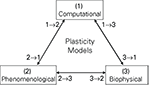Figure 1. Common motivations for plasticity models. The numbers denote affiliation of a model with respect to the three basic motivations, i.e., a model classified as 2 → 1 would be mostly phenomenologically based, but with some computational features. This classification is employed for the taxonomy of the models in Table 3.

Plasticity models can also be classified with respect to the mechanisms incorporated in them. Figure 2 contains a systematization of the mechanisms postulated in long-term plasticity. To enable a classification of the three types of model groups described earlier, some generalization is obviously needed, since usually only the biophysical models provide explicit statements about the underlying mechanisms, while other models state their mechanisms only implicitly and may also assume mechanisms inconsistent with biological evidence. Thus, this mechanism listing is neither comprehensive nor biophysically accurate. It simply resembles the most common denominator of effects found in various plasticity models, enabling a taxonomy of the whole model range expressed in Table 3 in Section “Model Comparison.”

FIGURE 2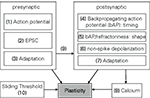Figure 2. Overview of mechanisms commonly included in plasticity models. The numbers are used in the taxonomy of the models in Table 3. A standard STDP (Song et al., 2000; Froemke et al., 2006) that computes the change in plasticity directly from the relative timing of pre- and postsynaptic spikes would be denoted by (1, 4). Some kind of presynaptic influence on the postsynaptic side (e.g., if a PSC raises the postsynaptic potential) is denoted by 9, a metaplastic sliding threshold by 10, etc.

On the presynaptic side, the most common mechanism included in models is a simple “time of event” of the presynaptic spike arriving at the synapse, denoted as “presynaptic action potential” in Figure 2. The next level of detail in presynaptic mechanisms of plasticity may be the explicit modeling of an EPSC or some derivation of it, with the waveform of the EPSC having influence on the shape of the plasticity function (Abarbanel et al., 2002; Shouval et al., 2002; Saudargiene et al., 2004). Another commonly included mechanism is presynaptic adaptation, i.e., some form of short term plasticity which governs how presynaptic spikes occurring in close temporal proximity to each other interact in their respective plastic influence on the synapse (Sjöström et al., 2001; Froemke et al., 2006; Shah et al., 2006; Lu et al., 2007; Zou and Destexhe, 2007).

On the postsynaptic side, the counterpart to the presynaptic spike arrival would be the backpropagating action potential (bAP), which according to most common STDP models signals the timing of an action potential at the soma back to the synapse. Thus, the complete classification of a simple STDP model (Song et al., 2000) would be “1, 4” which symbolizes the “relative-time-of-event” nature of STDP, i.e., the weight change is only driven by the pre- and postsynaptic timing difference. Complimentary to plasticity driven by the shape of the EPSC, there are also models which incorporate the exact shape of the bAP or the postsynaptic membrane characteristics (e.g., refractoriness) in computing their plasticity (Abarbanel et al., 2002; Shouval et al., 2002; Saudargiene et al., 2004; Badoual et al., 2006; Pfister et al., 2006; Shah et al., 2006). A model classified with non-spike depolarization would mean there is an explicit voltage driven mechanism on the postsynaptic side, i.e., the membrane voltage is not just assumed to convey the timing or shape of a bAP, but can in itself be altered (hyper- or depolarized with respect to the resting potential) to affect plasticity (Abarbanel et al., 2002; Clopath et al., 2010). Postsynaptic adaptation is also part of some models, mostly based on the decreased excitability of a neuron immediately after its action potential (Badoual et al., 2006; Froemke et al., 2006; Shah et al., 2006). However, there are also models which assume increased excitability (Pfister and Gerstner, 2006). Partially or wholly Ca2+-based models, such as (Shouval et al., 2002; Kurashige and Sakai, 2006; Shah et al., 2006) would be denoted by “Calcium” in Figure 2.

Metaplasticity can occur at various different organizational levels from synapse to network and express itself in various parameters of the involved neurons (Abraham, 2008). However, all models reviewed in this paper which touch on the subject of metaplasticity do so only in terms of the sliding threshold property separating LTD and LTP on a postsynaptic frequency scale, as introduced in the original BCM formulation (Bienenstock et al., 1982). Three major possible mechanisms for this sliding threshold can be discerned, i.e., direct influence on the plasticity parameters (Izhikevich and Desai, 2003; Benuskova and Abraham, 2007), a sliding adjustment of one of the postsynaptic state variables (Abarbanel et al., 2002), or a frequency threshold as a function of the mean Ca2+ elevation (Kurashige and Sakai, 2006). For Table 3, we classify a model as “10,” i.e., containing a sliding threshold, if this model either just derives a sliding threshold based on its original parameters (Izhikevich and Desai, 2003) or if it in addition contains an explicit mechanism adjusting this sliding threshold (Benuskova and Abraham, 2007).

### Local Correlation Plasticity and Corresponding Pre- and Postsynaptic Dynamics

#### Local correlation plasticity

A variety of experiments have shown the marked influence of membrane voltage on synaptic plasticity (Artola et al., 1990; Ngezahayo et al., 2000; Sjöström et al., 2001). On the other hand, spike timings as used in STDP protocols (Bi and Poo, 1998; Froemke and Dan, 2002) have to be detected locally by a synapse to trigger changes in its transmission properties. For this detection mechanism at the postsynaptic site, the membrane potential is a readily available candidate variable. One could assume that the membrane potential becomes progressively higher close to an postsynaptic AP, whereas after an AP it rises out of its hyperpolarization with an exponential curve (Koch, 1999). Thus, a read-out of the postsynaptic membrane potential at presynaptic spikes would lead to an STDP-like rule in this case (Brader et al., 2007).

Plasticity being bound to the co-occurrence of presynaptic and postsynaptic activity is also reminiscent of the BCM rule (Bienenstock et al., 1982), a well-established, rate-based learning paradigm with great experimental evidence (Dudek and Bear, 1992; Mayford et al., 1995; Wang and Wagner, 1999). In this rule, a presynaptic activity variable d(t) is multiplied with a shifted postsynaptic activity variable c(t) to define the change of synapse weight m: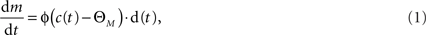where ΘM is an activity threshold and φ(.) represents a continuous non-linear function that changes sign at zero. The above formulation is somewhat reminiscent of the weight derivation in Abarbanel et al. (2002), where a product of pre- and postsynaptic activity results in the overall weight change. However, apart from the differing expression for pre- and postsynaptic activity, the model of Abarbanel et al. (2002) derives its weight as a competition between both activities, whereas the above equation simply describes the combined actions of both pre- and postsynaptic state variables.

From our point of view, the postsynaptic activity c(t) is the membrane potential and the threshold ΘM is a voltage threshold. This recasting is partially inspired by earlier work of our research group on a membrane voltage-based plasticity rule for PCNN image processing, which takes on a similar form (Schreiter et al., 2002). As well, a voltage threshold for separating LTD and LTP induction is supported by the results reported in Artola et al. (1990), Fusi et al. (2000), and Ngezahayo et al. (2000). Formulating BCM using spike time-dependent instead of purely rate-dependent components is also beneficial in terms of its resultant computational capability (Toyoizumi et al., 2005).

The presynaptic activity d(t) still needs to be defined in our framework. Because the plasticity mechanism needs to have direct access to the membrane potential, it can only work at the postsynaptic site; thus, also the presynaptic read-out has to occur there. Under this assumption, a candidate read-out variable is the conductance change of postsynaptic ion channels that is triggered by presynaptic neurotransmitters, released in reaction to presynaptic spikes. With this conductance change, the complete LCP rule reads as follows: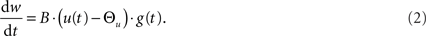In this equation, w(t) is the weight of the synapse, g(t) is the conductance of presynaptically activated channels, u(t) is the membrane potential and Θu is the threshold between potentiation and depression. Besides the recasting of the pre- and postsynaptic variables, the main difference between the original BCM formulation and Eq. 2 is that the non-linear function φ() relating postsynaptic activity to plasticity is replaced by a linear scaling with a proportionality constant B. However, as we show later, this linear dependence of plasticity on voltage translates to a non-linear dependence on the postsynaptic rate which is compatible with the original BCM intentions.

We assume that the postsynaptic membrane potential eliminates the block of NMDA receptors, subsequently releasing Ca2+ and plastically changing the synaptic weight (Senn, 2002; Shouval et al., 2002). Thus, B should be defined in units of 1/(As), which has the effect of “canceling” the Ca2+ charge unit, allowing us to arrive at a dimensionless synaptical weight as required for comparison with most of the experimental results (Bi and Poo, 1998; Sjöström et al., 2001; Froemke and Dan, 2002). The postsynaptic expression B · (u(t) − Θu) thus results in units Ω/s, which could be interpreted as the opening/closing speed of the Ca2+ channel (i.e., its resistance change), consequently linking LTP/LTD of our expression with the rate of Ca2+ release (Aihara et al., 2007).

The behavior of the LCP rule crucially depends on the models for the neuron and the synaptic conductance. In the following two sections, we introduce simple models for both which are suited for analyzing the principal rule characteristics as well as for quantitatively deriving the weight changes in response to experimental protocols. In Section “Neuron Dynamics: Leaky Integrate-and-Fire,” we extend the neuron model to a more realistic LIAF formulation.

#### Synapse dynamics

For the synaptic conductance g(t), we use an exponential decay function in response to each presynaptic spike, which is a simplification of the synapse model described in Gerstner and Kistler (2002):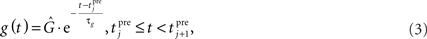where τg is the time constant of the decay and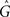is the amplitude of the response. Setting the presynaptic conductance to its maximumat the beginning of each presynaptic pulse effectively makes the learning carried out in the LCP rule history-independent. In standard STDP terminology, this would be equivalent to a presynaptic nearest-neighbor interaction (Morrison et al., 2008).

This can be extended to a presynaptic all-to-all interaction via the integration of all presynaptic spikes in the current weight dynamics. Mathematically, this amounts to replacing the constant conductanceby a spike-varying conductance Gj as follows: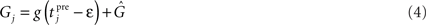Thus, the conductance amplitude immediately before the current presynaptic spike is contained in the new amplitude, conserving the spiking history at the synapse, leading to presynaptic all-to-all interaction (Morrison et al., 2008).

#### Neuron dynamics: spike response model

For the membrane potential, we choose a spike response neuron model (Gerstner and Kistler, 2002), consisting of a Dirac function for the action potential and an exponential decay function for the hyperpolarization after firing: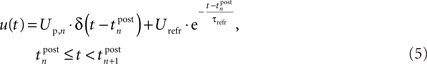Thereby,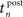denotes the n-th postsynaptic spike, Up,n is the area under the pulse curve and Urefr < 0 and τrefr are the amplitude and time constant of hyperpolarization. Note that u(t) reflects deviations of the membrane potential from its equilibrium; a resting potential could be added when adjusting the voltage threshold Θu accordingly.

Additionally to the hyperpolarization of the membrane potential, we added an attenuation of the spike amplitude during refractoriness to model the decreased excitability during this period (Shah et al., 2006) and the frequency-dependent attenuation of both amplitude and duration found in Froemke et al. (2006) and Tanaka et al. (1991). Therefore, we used a simple formulation that weights the amplitude of the n-th postsynaptic spike with the amount of membrane hyperpolarization directly before the spike: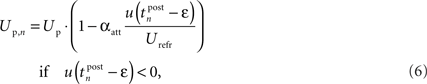where Up is a fixed parameter that determines the maximum amplitude of the postsynaptic pulse. Please note that in contrast to the similar postsynaptic attenuation of Froemke et al. (2006), we did not introduce a new time constant for this saturation effect. This addition has significant influence on the rule behavior in triplet experiments, as we will show in Section “Simulation Results.”

The biophysical motivation for this influence of the postsynaptic attenuation on plasticity is somewhat speculative. Shah et al. (2006) contain some references to the attenuation of BPAPs from which they derive a modeling argument for including this attenuation effect in plasticity. However, we have only found one reference (Froemke et al., 2006), where both postsynaptic adaptation and its influence on plasticity is shown. In Figure 7 of Froemke et al. (2006), it is shown that when chemically blocking postsynaptic attenuation in plasticity experiments which involve several postsynaptic spikes, the resulting plasticity at the synapse is actually replicated better by naïve STDP than by their attenuation model. For the unaltered preparation, the opposite is shown, i.e., their attenuation model captures the data significantly better than STDP without attenuation. So at least for the preparation of Froemke et al. (2006), a postsynaptic attenuation is proven which has the proposed influence on plasticity, i.e., for closely following postsynaptic spikes, the effect of later spikes on the overall plastic change at the synapse diminishes. Figure 9 in Section “Simulation Results” shows the correspondence of the attenuation of Eq. 6 with the experimental data of Figure 7B of Froemke et al. (2006).

#### Neuron dynamics: leaky integrate-and-fire

In the previous section, we have introduced a simple neuron for the LCP rule. Thereby, we have neglected important ingredients of neuronal signal transmission. In the following, we therefore extend the neuron model, incorporating the influence of a presynaptic spike on the postsynaptic membrane potential. This influence can be determined by the current flow through the cell membrane that is triggered by presynaptic neurotransmitter release. The current can be modeled similar to Eq. 2 as: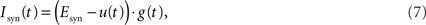where Esyn is the reversal potential of that channel, which is approx. 70 mV above resting potential for excitatory synapses (Gerstner and Kistler, 2002). The conductance g(t) is defined as in Eq. 3. Because we are only interested in sub-threshold variations of the membrane potential, we use the approximation Esyn >> u(t), so that the time course of Isyn(t) is only determined by g(t).

To calculate the influence of the synaptic current on the membrane potential, we use a LIAF neuron, which is defined by the differential equation: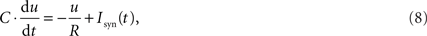where C and R are the capacitance and resistance of the membrane that together result in the membrane time constant τrefr = C · R, which was already used in the SRM neuron model in Eq. 5. As for the learning rule in Eq. 2, we assume a resting potential of 0 V. We then have to solve the following differential equation: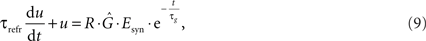whereby we assume, without loss of generality, that the presynaptic pulse occurs at t = 0. Furthermore, we only regard the case τrefr ≠ τg, which is justified by the parameter sets in use (cf. Table 4).

We suppose that the solution has the form: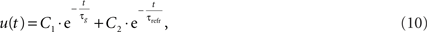with constants C1 and C2. Calculating these constants results in the solution: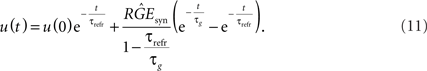We want to dimension the unknown amplitude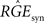by the amplitude of the postsynaptic potential UPSP that results from a presynaptically triggered current injection at postsynaptic rest (u(0) = 0). Calculating this maximum from the necessary condition du/dt = 0 results in: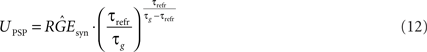The final explicit formulation for u(t) then is: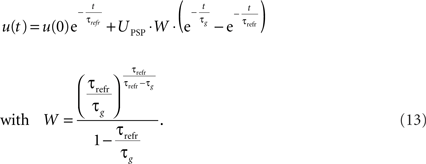We will use these equations for the simulations with PSP amplitude.

The remainder of the neuron behavior is the same as for the SRM model: at a postsynaptic spike time, a Dirac pulse with area Up is generated. Afterwards, the membrane potential u is reset to the refractory amplitude Urefr from where it evolves according to Eq. 13. In the case of no presynaptic activity, this results in an identical time course compared to the SRM model.

In the standard configuration, the LIAF neuron does only generate postsynaptic spikes at the times defined by the experimental protocol. As an exception, for the correlated activity setting in the Dudek and Bear (1992) protocol (cf. Benchmark Experiments), the LIAF neuron may pulse on its own. Thereby, a spike is emitted if the membrane potential exceeds a noisy threshold Θfire (Gerstner and Kistler, 2002). The value of Θfire is changed at each presynaptic spike, drawing it from a Gaussian distribution with mean 2 · UPSP and standard deviation 5% of the mean.

#### Summary of LCP versions

As stated in the Section “Introduction,” two versions of the LCP learning rule will be analyzed in Section “LCP with SRM Neuron” respectively Section “LCP with LIAF Neuron.” Both versions use the LCP rule as defined in Eq. 2, the PSC of Eq. 3 and the attenuation of postsynaptic action potentials in Eq. 6. The main difference between both versions is the type of neuron used to construct the postsynaptic membrane voltage profile u(t), i.e., the SRM of Section “Neuron Dynamics: Spike Response Model” or the LIAF of Section “Neuron Dynamics: Leaky Integrate-and Fire”:

• The first version of the LCP rule, referred to as LCP with SRM, employs the definition of the membrane potential in Eq. 5.

• The second version of the LCP rule, referred to as LCP with LIAF, employs the definition of the membrane potential of Eq. 13 and the corresponding UPSP of Eq. 12. The all-to-all presynaptic PSC interaction of Eq. 4 is also part of this version of the rule.

The fit of both versions of the LCP rule to the experimental data in Sections “LCP with SRM Neuron” and “LCP with LIAF Neuron” was estimated using the normalized mean-square error E defined in Pfister and Gerstner (2006). In summary, the analysis of the two versions of the LCP rule uses the symbols given in Table 2.

TABLE 2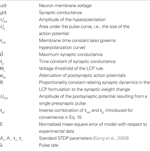Table 2. Listing of symbols used in the analysis of LCP with SRM in Section “LCP with SRM Neuron” and LCP with LIAF in Section “LCP with LIAF Neuron.”

## Results

### Model Comparison

Table 3 gives a comparison of a broad selection of plasticity models. Emphasis is laid on reproduction of biological experiments and generalization capability. In this context, the number of parameters in a model is included in Table 3 to identify which models make parsimonious use of parameters in fitting experiments. Possible overfitting problems can thus be identified (Wei, 1975) and a rough comparison of computational effort in simulating a given model is possible. The performance of the models with respect to the experiments is classified with symbols “f,” “h,” “fc,” “hc,” “u,” “n,” and “?,” with their meaning given in the caption of Table 3. The comparison is started with the standard exponential formulation of STDP (Song et al., 2000), since STDP experiments (1) are replicated by most of the models in the table and can thus serve as a basis for the assessment of those models. Also, STDP should serve as Occams razor for more elaborate models, i.e., whether those models are able to replicate more phenomena than STDP. The detailed discussion of all reviewed models is given in the Appendix.

TABLE 3

To complete Table 3, an assessment of both LCP with SRM and LCP with LIAF is given, with detailed treatment of both model versions deferred to Section “LCP with SRM Neuron” and Section “LCP with LIAF Neuron.” LCP with SRM is able to reproduce basic STDP (1) behavior (Figure 4). The LTD half of frequency-dependent STDP (2) is reproduced (Figure 10B), but the LTP half lacks the weight increase for high frequencies. Experiments (3, 4, 7) are reproduced (Figures 7C, 8A, and 6B, respectively), but the parameter settings across the experiments are slightly inconsistent. LCP with SRM cannot reproduce the standard rate experiment (6) satisfactorily for any of the assumptions regarding the activity on the postsynaptic side. With respect to burst (5) plasticity, LCP with SRM basically behaves as conventional STDP (Figure 14A), not able to show the plasticity behavior found in this experiment. Both versions of the LCP rule can replicate the LTD/LTP threshold in the voltage control experiment (8) (Figure 12C), but not the lower bound of the LTD window, so a “h” classification is given. LCP with LIAF is able to reproduce almost all experiments (see Table 4 and Figures in LCP with LIAF Neuron). The exceptions are bursts (5), where either the pre-burst-post or the post-pre-burst case can be reproduced (Figures 14B,C), and the limit just discussed for experiment (8). In the next section, detailed results on LCP with SRM and the reasoning corresponding to the above assessment will be given.

TABLE 4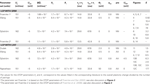Table 4. Parameters used for experiment reproduction. Normalized errors (E) are given for all experiments for which data points with standard errors were available. If several Figure numbers are given in a row, the corresponding normalized error is calculated over all experiments in the Figures for the parameter set in the row (which may deviate from the parameter set used in the Figures).

### LCP With SRM Neuron

#### Analysis results

Figure 3 shows the principal operation of LCP with the SRM neuron. Unlike spike-based learning rules, there is a continuous update of the synapse weight whenever the membrane potential deviates from the voltage threshold Θu during presynaptic activity.

FIGURE 3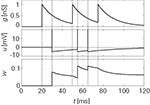Figure 3. Progression of the conductance g, the membrane potential u and the synapse weight w for a sample spike pattern. Units were chosen to be in a biologically realistic range: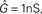B = 1/(1 pC). The remainder of the neuron and synapse parameters were taken from the Froemke 1 parameter set listed in Table 4.

For the SRM neuron model, there is a single mechanism each for LTP and LTD: LTP is triggered at each postsynaptic spike. Due to the idealized pulse shape, the conductance variable g(t) is sampled at each postsynaptic spike time. Integrating Eq. 2 expresses this in the formula: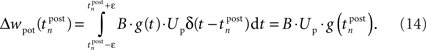This mechanism is equivalent to an iterative implementation of LTP in pair-based STDP models (Pfister and Gerstner, 2006). LTD, on the other hand, is mediated by continuous integration of the presynaptic conductance in the refractoriness period after a postsynaptic spike. Thus, the LTD mechanism always acts if pre- and postsynaptic spikes occur in close temporal proximity. Additionally, LTD is active if the voltage threshold is above resting potential, i.e., Θu > 0. In the interval (t0, t1) between two consecutive spikes, the resulting LTD weight change can be calculated based on Eq. 2 from the starting values g(t0) and u(t0):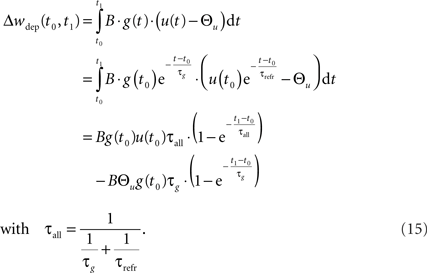In the final weight change, potentiation may override this depression if there is presynaptic activity at a postsynaptic spike, i.e., if a presynaptic spike occurs shortly before a postsynaptic spike. This directly leads to the temporal asymmetry seen in pair-based STDP rules. We show this by calculating the weight change induced by a spike pairing with time difference Δt = tposttpre. For a pre-post pairing, the weight is at first potentiated by the postsynaptic spike and then depressed due to refractoriness. The amount of potentiation can be calculated from Eq. 14, with the conductance at the postsynaptic spike being equal to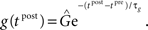The amount of depression that accumulates up to a time t > tpost is determined by Eq. 15, with the depression starting at t0 = tpost and ending at t1 = t, so that g(t0) = g(tpost) as above and u(t0) = Urefr. Combining the potentiation and depression parts results in: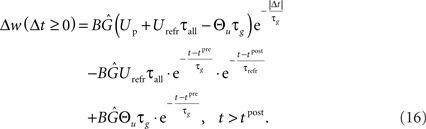In contrast, for a post-pre pairing no potentiation occurs at all, because there is yet no presynaptic activity present at the postsynaptic spike. For the same reason, depression starts to act only from the presynaptic spike onward, i.e., t0 = tpre. Thus, the starting values are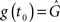and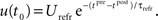Again from Eq. 15, the resulting weight change calculates as: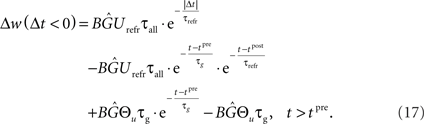Using Eqs. 16 and 17, our LCP with SRM can be directly mapped to pair-based (nearest-neighbor) STDP rules (Morrison et al., 2008). Thereby, we set Θu = 0. Then, a spike pairing with time difference Δt = tposttpre results in the time-continuous weight change function: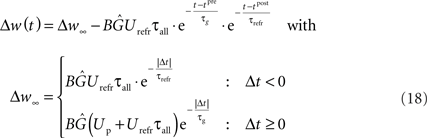As denoted, the solution of the integral can be split into a time-invariant term Δw that depends on the order of the spike pairing, and an additional time-dependent term that diminishes for t → ∞. For the low pairing frequency used in standard STDP experiments (Bi and Poo, 1998; Froemke and Dan, 2002), the resulting weight change is well approximated by the term Δw. This term equals the exponential time window of pair-based STDP, as is shown in Figure 4.

FIGURE 4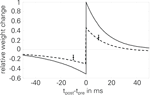Figure 4. Normalized STDP window of LCP with SRM: analytical value Δw (solid line) and simulation results (circles, 60 pairings at 1 Hz, protocol of Bi and Poo, 1998; Froemke and Dan, 2002). STDP parameters are taken from Froemke and Dan (2002) and corrected by the number of repetitions, cf. Froemke 1 parameter set in Table 4. Error bars are measurements from Wang et al. (2005), as extracted by Pfister and Gerstner (2006); dashed line: simulation results for Wang parameter set, used to account for the smaller weight changes found throughout the experiments in Wang et al. (2005), as stated there. Normalized error in this case: E = 0.92.

From this correspondence, the parameters of LCP with SRM can be directly derived from the parameters of the exponential STDP time window: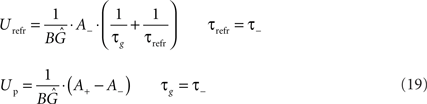In these correspondences, the parameters A(<0), τ and A+, τ+ denote the amplitude and time constant of LTD and LTP, respectively (Song et al., 2000). To arrive at biologically realistic membrane voltage values, we calculate the amplification factor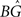such that the refractory amplitude Urefr equals −5 mV (Koch, 1999).

It is important to note that the parameters of LCP with SRM, being strongly linked to biophysical parameters, take on biologically realistic values when they are derived from measured STDP parameters. The conductance time constant τg equals the STDP time constant for LTP, which is in the order of 20 ms, a value compatible with NMDA synapse conductance changes (Gerstner and Kistler, 2002; Badoual et al., 2006). In contrast to (Pfister et al., 2006), this time constant is chosen different from τmem, since those characteristics are not necessarily correlated (Koch, 1999; Senn, 2002). The STDP time constant for LTD equals the membrane time constant τrefr, which was found to be in the order of 10–40 ms (Koch, 1999). As can be derived from Eq. 19, the STDP parameters A+, τ+ and A, τ set the relation between Up and Urefr. For our assumption, Urefr = −5 mV, the STDP parameters of Froemke and Dan (2002) result in Up = 151 μVs (cf. Froemke 1 parameter set in Table 4), corresponding to a rectangular pulse of length 2 ms and height 75 mV, which are reasonable values for an action potential (Koch, 1999).

The increase in LTD with the amplitude of the refractoriness, Urefr, as evident from Eq. 18, is in direct contrast with Pfister et al. (2006), where LTD increases with a decrease in refractoriness. The motivation given in Pfister et al. (2006) is that an additional refractoriness has to be introduced through the learning rule to block a presynaptic spike which is not sufficiently suppressed by the refractoriness. In contrast, the LTD mechanism of LCP with SRM could be interpreted as enhancing the effectiveness of the presynaptic pulse, i.e., a given presynaptic PSC is not allowed to simply spend itself against the refractoriness as in Pfister et al. (2006), which would be wasteful from an information- and energy-conserving point of view.

Besides the temporal asymmetry seen in STDP, the LCP rule also exhibits a close similarity to the BCM model in its formulation, as was discussed in Section “Local Correlation Plasticity.” It is therefore interesting to check whether LCP with SRM can be mapped to the original BCM rule as well. Especially, relating the voltage threshold Θu in the LCP rule to the frequency threshold ΘM in the BCM rule could open BCM arguments on rate-based metaplasticity (Abraham et al., 2001) to our rule, as well as providing a possible link to voltage-based expressions of metaplasticity (Ngezahayo et al., 2000). For deriving such a relationship, we use an analysis similar to that in Izhikevich and Desai (2003). We start out with the general derivation for the weight change of a single pair of spikes as done above, and again assume low pairing frequency, so omit the terms dependent on t. But in contrast to the STDP derivation, we treat Θu as non-zero parameter. The resulting expression for LTP is as follows: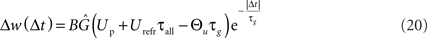and for LTD: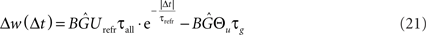Assuming a Poisson process for postsynaptic spike times, the expected weight change Δw(λ) for a single presynaptic spike and a postsynaptic firing rate λ can be explicitly derived. This is done by integrating the product of the weight change as a function of time Δt and the Poisson probability density pt) = λ· exp(−λ· Δt) separately for LTP and LTD. Adding both integrals results in the overall weight change (Izhikevich and Desai, 2003):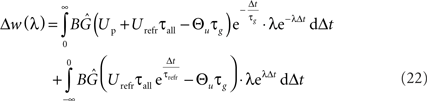Carrying out this integration results in the following expression: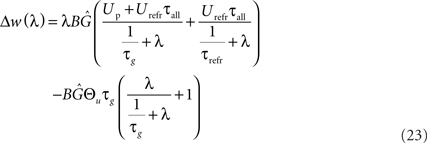The first part of the above expression is basically the same STDP-BCM translation as in Izhikevich and Desai (2003), with the standard STDP parameters substituted as in Eq. 19. The second expression causes the entire weight change curve to shift upwards or downwards, dependent on Θu, while the additional dependence on λ can be neglected for a small λ, only causing an increase in the slope of the curve for a high λ (>1/τg), thus letting the curve crossover from LTD to LTP slightly earlier. Consequently, as is evident from Eq. 23 and the curves in Figure 5A, the voltage threshold for plasticity introduced in the LCP rule can be related to the sliding frequency threshold found in the context of rate-based induction protocols (Mayford et al., 1995; Wang and Wagner, 1999; Abraham et al., 2001). Ngezahayo et al. (2000) show that such a voltage threshold separating LTP and LTD exists and that it can be metaplastically adjusted. This adjustment changes the ratio and crossover point between LTD and LTP in a fashion qualitatively compatible with the original formulation for the sliding frequency threshold (Bienenstock et al., 1982; Ngezahayo et al., 2000). An interesting observation in Figure 5A is that for low frequencies, contrary to most BCM formulations that assume no plasticity change, the plasticity can exhibit an offset toward either LTD or LTP, depending on the voltage threshold. This kind of voltage offset is very similar to the results reported in Sjöström et al. (2001), where a depolarization causes an offset toward LTP at low frequencies, while a hyperpolarization results in enhanced LTD at low frequencies. Also, similar to the theory of Beggs (2001), Figure 5A shows that a vertical shift of the plasticity curve via a voltage offset results in a horizontal shift of the frequency threshold.

FIGURE 5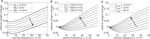Figure 5. Plot of the analytical BCM expression of Eq. 23 for sweeps of different variables. The standard parameter set (Froemke 1 in Table 4: Θu = 0 mV, Up = 151 mV·ms, Urefr = −5 mV) is always denoted by the broken line. (A) sweep of Θu; (B) sweep of Up; (C) sweep of Urefr.

In contrast to Θu, a sweep of the two amplitude parameters Up and Urefr affects only the second threshold from LTD to LTP, while the plasticity for low frequencies remains unchanged (Figures 5B,C). Both have a very similar effect of lowering/raising this frequency threshold while changing the overall shape of the curve from a “U” shape to an almost linear characteristic.

#### Simulation results

In a first test related to the BCM discussion of the previous section, we use the correlated rate protocol (7) to verify that LCP with SRM follows the simplifications of Eq. 23. Since the experiments of Sjöström et al. (2001) employ a different cell preparation and produce, e.g., zero potentiation for a standard pre-post pairing protocol in contrast to the strong LTP of conventional STDP experiments (Bi and Poo, 1998; Froemke and Dan, 2002), a parameter set different from the one of Froemke and Dan (2002) is used in Figures 6 and 10. This parameter set has been chosen to approximate the STDP window of Sjöström et al. (2001), see also the motivation in Figure 13.

FIGURE 6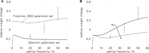Figure 6. Correlated rate protocol (7); (A) standard nearest-neighbor STDP; (B) LCP with SRM without and with attenuation (αatt = 0, αatt = 0.8), using Sjöström parameter set (see Table 4). Error without attenuation: E = 3.2. Error bars denote experimental data by Sjöström et al. (2001).

Figure 6 shows the corresponding weight changes for a standard STDP model (A) and LCP with SRM (B). The STDP model exhibits only a slight dependence on the pairing frequency, producing either LTP or LTD throughout the frequency range, dependent on the parameter set. This is in contradiction to the experimental results of Sjöström et al. (2001) that show depression below approx. 35 Hz and potentiation for frequencies above only (cf. error bars in Figure 6). In contrast, LCP with SRM is qualitatively compatible with the experimental data, exhibiting a transition from LTD to LTP at approx. 25 Hz. However, attenuation of postsynaptic action potentials would add a source of depression at higher frequencies that prevents a transition to LTP. Thus, this addition is not compatible with the experimental protocol.

We further tested LCP with SRM for spike triplet experiments (3). Therefore, we used the STDP parameters given in Froemke and Dan (2002) to compare to their triplet measurements. Figure 7 shows the original data points (A) together with predicted weight changes for LCP with SRM (B and C) as well as for a standard pair-based STDP rule (D). LCP with SRM (B) and STDP (D) show almost the same behavior, which is also in good agreement with the measurements. Only for the post-pre-post triplet (lower right quarter), qualitative differences are visible: whereas the models predict potentiation for small time differences t1 and t2, the measurements show depression. Introducing the attenuation of closely following postsynaptic spikes (αatt = 0.8), the amount of potentiation is significantly reduced in this case, so that depression dominates as in the measurements, see Figure 7C. Thus, even LCP with SRM can fully reproduce these triplet experiments, contrasting, e.g., the more extensive models by Badoual et al. (2006). If postsynaptic attenuation is switched off, our model is also somewhat compatible with the triplet experiments of Wang et al. (2005) (cf. circles in Figure 7B), slightly outperforming standard STDP (cf. error measures in Figure 7). As discussed in Section “Benchmark Experiments,” a full compatibility for both triplet results cannot be achieved in a single model due to the fundamental difference in experimental behavior.

FIGURE 7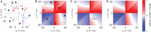Figure 7. Triplet protocol (3). Upper left part: 2 presynaptic, 1 postsynaptic spike; lower right part: 1 presynaptic, 2 postsynaptic spikes; (A) experimental data of Froemke and Dan (2002)(B) LCP with SRM, using parameters from Froemke and Dan (2002), as listed in Table 4 (Froemke 1 parameter set); circles: data by Wang et al. (2005) (error to Wang data for LCP with SRM and Wang parameter set: E = 10.2); (C) LCP with SRM with postsynaptic attenuation (αatt = 0.8); (D) standard STDP rule with same parameters, for comparison (error to Wang data with Wang parameter set: E = 12.9).

Regarding the quadruplet protocol of Wang et al. (2005), pair-based STDP rules fail to reproduce the experimental results, see (Pfister and Gerstner, 2006) and Figure 8B. In contrast, LCP with SRM can reproduce the dependency of weight change on the time interval T, see Figure 8A. To account for the reduced potentiation for big time differences T compared to standard STDP, we used an increased voltage threshold in the Wang parameter set (Θu = 0.5 mV, cf. Table 4). Like for the triplet experiments, LCP with SRM can not reproduce the Wang data if attenuation of postsynaptic spikes is activated, as is shown in Figure 8A.

FIGURE 8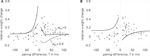Figure 8. Quadruplet protocol (4). Single data points in the diagrams extracted from Wang et al. (2005), points with error measures as given in Pfister and Gerstner (2006), based also on Wang et al. (2005). (A) LCP with SRM with (dashed) and without (solid) postsynaptic attenuation, Wang parameter set (see Table 4); E = 5.6 without attenuation; (B) standard STDP rule with same parameter set, E = 17.8.

These observations casts doubt on the validity of the postsynaptic attenuation in Eq. 6. To show the necessity of the attenuation at least in the scope of the experiments of Froemke and Dan (2002) and Froemke et al. (2006), Figure 9 replicates the experiment of Figure 7B in Froemke et al. (2006), where postsynaptic attenuation and its influence on plasticity is explicitly tested. As is shown, LCP with SRM replicates both the standard experiment and the one with suppressed attenuation satisfactorily for the corresponding settings of αatt. The LCP rule produces somewhat too much potentiation for 4 and 5 postsynaptic APs, which could be due to the fact that the synapses in the preparation of Froemke et al. (2006) seem to exhibit a hard weight bound at about 60% potentiation, i.e., any hypothetical further weight increase is checked by the saturation of the weights. As stated earlier, such a saturation is not included in the LCP model.

FIGURE 9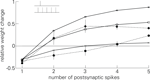Figure 9. Plasticity curves for the post-pre-post-burst protocol of Figure 7B of Froemke et al. (2006), change in EPSP for the standard experiment (dashed line, filled circles) and with suppressed postsynaptic attenuation (dashed line, diamonds), including error bars This experiment for LCP with SRM (parameters taken from Froemke et al., 2006), compare (Froemke 2 parameter set in Table 4) and activated attenuation (crosses, αatt = 0.8) or deactivated attenuation (stars, αatt = 0); For comparison: standard STDP (empty circles), same parameters as LCP with SRM.

#### Limits of LCP with SRM

In the previous section, we have shown that LCP with SRM can reproduce a variety of experimental protocols, but only with conflicting settings for the attenuation of postsynaptic spikes: whereas this attenuation is necessary for replicating the data by Froemke and Dan (2002) for the triplet protocol (3), it leads to incompatible results in the quadruplet protocol (4) and the correlated rate protocol (7).

As a further limitation, LCP with SRM has some difficulties in reproducing frequency-dependent STDP (2), even without postsynaptic attenuation, cf. Figure 10B. Still, it is compatible with the experimental data at least qualitatively, in contrast to standard STDP, cf. Figure 10A. However, LCP with SRM fails to account for the increasing potentiation for pre-post pairings.

FIGURE 10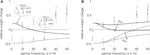Figure 10. Frequency-dependent STDP (2); error bars denote measurement data of Sjöström et al. (2001) . Dashed curves and error bars: post-pre pairings, solid lines and error bars: pre-post pairings. Error bars at 40 and 50 Hz were slightly moved apart to make them distinguishable. (A) standard STDP [the upper curve (pre-post pairings) is also shown qualitatively in Standage et al. (2007)], (B) LCP with SRM neuron for αatt = 0 and αatt = 0.8. Sjöström parameter set as listed in Table 4. Normalized error without attenuation: E = 6.8.

### LCP with LIAF Neuron

In the following, we want to investigate whether the second LCP version of Section “Summary of LCP Versions,” i.e., extending LCP with the LIAF neuron of Section “Neuron Dynamics: Leaky Integrate-and-Fire,” can overcome the current limits of LCP as evident from Section “Limits of LCP with SRM.” Therefore, we test LCP with LIAF on a variety of experimental protocols. We start with results for the standard rate protocol (6) to illustrate the effects of the extensions.

In the LCP rule, a weight change can only occur if presynaptic activity coincides with the postsynaptic membrane potential u deviating from the voltage threshold Θu. Furthermore, the direction of the weight change is directly related to the sign of the deviation (u − Θu). In protocols with only presynaptic stimulation, a transition from depression to potentiation is thus impossible if the presynaptic activity has no influence on the postsynaptic membrane potential, as in LCP with SRM. As a consequence, LCP with SRM, like standard STDP rules, can not account for the standard rate (6) results without making strong assumptions on postsynaptic firing, like postsynaptic firing rate being proportional to presynaptic firing rate (Izhikevich and Desai, 2003), or even single-spike correlations (Beggs, 2001; Standage and Trappenberg, 2007). This is documented in Figure 11C.

FIGURE 11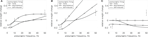Figure 11. Standard rate protocol (6). Error bars denote measurement data by Dudek and Bear (1992). (A) LCP with LIAF for different postsynaptic spike settings, nearest-neighbor mode; Dudek parameter set (cf. text and Table 4); errors for the postsynaptic settings: none: E = 1.5, spontaneous: E = 10.7, self-induced: E = 2.0. (B) Same as (A), but with all-to-all interaction and postsynaptic attenuation (αatt = 0.8); (C) nearest-neighbor STDP model with same parameters for comparison.

In contrast, the postsynaptic potential added by the LIAF neuron depolarizes the membrane voltage with increasing presynaptic stimulation frequency, so that the membrane voltage can cross the voltage threshold Θu, leading to potentiation even in the absence of postsynaptic spikes. As Figure 11A shows, this leads to a transition from weight depression to potentiation, which is what Dudek and Bear (1992) found experimentally. The point of transition from depression to potentiation is dependent on the STDP time constants. To account for the relatively low transition frequency in the data by Dudek and Bear (1992), we therefore used the Sjöström parameter set as reference, because it has longer time constants. Even though the induction protocol in Dudek and Bear (1992) was much stronger than for spike-based experiments (900 pulses compared to, e.g., 60 pairings), the resulting weight changes of both are in the same order of magnitude. To account for this difference, we divided the weight change amplitudes by a factor of 900/60 = 15.

Besides the non-spiking postsynaptic side investigated above, we also tested the uncorrelated and correlated spiking settings as discussed in Section “Benchmark Experiments” by triggering postsynaptic spikes and membrane potential resets at the given times, while keeping the influence of presynaptic spikes on the membrane via postsynaptic potentials. Both protocols result in additional depression because of the resetting and hyperpolarization of the membrane potential. However, the initial results are not changed qualitatively. As an aside, the depression introduced by uncorrelated spiking is almost frequency-independent (cf. Figure 11A), and can be compensated, e.g., by increasing the PSP (error for UPSP = 2.5 mV: E = 1.9, not shown).

Introducing presynaptic all-to-all interaction cancels the LTP saturation in nearest-neighbor mode, as Figure 11B shows. This is caused by the accumulation in the presynaptic activity g(t), which counterbalances the increased leakage at higher membrane potentials, leading to a linear dependence of potentiation and presynaptic frequency in a broad range for uncorrelated and no postsynaptic spiking. As a consequence, weight change amplitudes would have to be reduced to at least partly account for the experimental results. As an exception, correlated firing still results in significant depression at higher frequencies, leading to compatible results with the parameter set in use (E = 1.6).

As Figure 11C shows, pair-based STDP consistently fails to account for the experimental results, irrespective of the postsynaptic spike setting.

For the quadruplet protocol (4), the LCP rule also benefits from the additions of the LIAF model. As Figure 12A shows, using all-to-all presynaptic interaction results in higher LTP for small positive pairing differences T, which is more compatible with the experimental data. Furthermore, in this case, the depression effect due to postsynaptic attenuation can be compensated by introducing PSPs, as is evident from Figure 12B.

FIGURE 12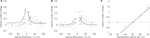Figure 12. (A, B); Quadruplet protocol (4) using LCP with LIAF: (A) same parameters as in Figure 8B, but all-to-all interaction (Wang parameter set in Table 4), E = 1. 9; (B) all-to-all interaction, with attenuation (αatt = 0.8), which is compensated by PSP influence: UPSP = Θu = 2 mV; E = 1.2. (C) Voltage control experiment (8). Error bars denote data for unconditioned synapse by Ngezahayo et al. (2000). Froemke 1 STDP parameters with downscaled weight amplitudes were used, and the voltage threshold was shifted according to the experimental data (see Ngezahayo parameter set in Table 4). Normalized error: E = 177.

Local correlation plasticity with SRM failed to reproduce the correlated rate experiment (7) when postsynaptic spikes were attenuated. Using the postsynaptic potential and the presynaptic all-to-all interaction counterbalances the negative attenuation effect, as is shown in Figure 13B. The postsynaptic potential leads to increased potentiation already for small frequencies. Therefore, we had to increase Θu to make the overall response negative. At high frequencies, the all-to-all interaction leads to increased postsynaptic potentials, which in turn flattens out the hyperpolarization after postsynaptic spikes and thus compensates for the attenuated spike amplitudes in this regime. Due to the postsynaptic potential the LTP part of the STDP modification window is distorted, as Figure 13A shows. By coincidence, this additional depression was also measured in Figure 2D of Sjöström et al. (2001).

FIGURE 13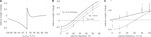Figure 13. Experiments of Sjöström et al. (2001) using LCP with LIAF and Sjöström parameter set (cf. Table 4). (A) resulting STDP window; (B) correlated rate protocol (7), E = 6.3; (C) Frequency-dependent STDP (2), E = 1.6. Total error: E = 2.9.

As can be seen when comparing the analytical expression of Figure 5A and the simulation of Figure 13B, the qualitative plasticity behavior caused by a Θu ≠ 0 corresponds to the analytical derivation, but the absolute value of Θu necessary to effect a certain behavioral change differs. This is due to the fact that Θu works mainly as an offset to the exponential refractoriness, which is assumed to extend to infinity in the analytical derivation. Thus, the overall area of the refractoriness and consequently the effect of Θu is larger in the analytical expression compared to the simulation, where the refractoriness is cut off with the next pulse. Correspondingly, Θu has to have higher absolute values in the simulation to achieve the same kind of effect.

The increased potentiation due to accumulated presynaptic conductance (all-to-all interaction) and its influence on membrane voltage via postsynaptic potentials also leads to more potentiation at high frequencies in the frequency-dependent STDP experiment (2), despite attenuation of postsynaptic spikes, cf. Figure 13C. Consequently, LCP with LIAF leads to steadily increasing LTP with pairing frequency for both pre-post and post-pre pairings, which is more consistent with the experimental data than LCP with SRM (see Figure 10B).

Figure 12C investigates the behavior of LCP with LIAF with respect to the voltage control experiment (8). Since the LCP rule contains only a single voltage threshold, whereas the experimental results in Artola et al. (1990) and Ngezahayo et al. (2000) suggest two thresholds, only the crossover from LTD to LTP is replicated, not the diminishing LTD below a certain membrane voltage.

However, if we rather take the LCP voltage threshold as a parameter and interpret the postsynaptic voltage clamp as activity level (i.e., equivalent to the time-averaged postsynaptic frequency, see also the discussion in Ngezahayo et al., 2000), some similarity can still be observed to the plasticity reported in Ngezahayo et al. (2000). As seen in Figure 5A, a decrease in Θu shifts the lower (i.e., LTD) threshold to higher frequencies, while at the same time transferring the LTP threshold to lower frequencies. So the curve for a depressed synapse in Ngezahayo et al. (2000) with an almost flat part followed by a single threshold for LTP could be approximately reproduced by a low Θu, while a high positive Θu acts to widen the LTD portion of the plasticity curve in both directions, similar to the potentiated synapse of Ngezahayo et al. (2000).

We further tested LCP with LIAF with the presynaptic burst protocol (5). Experimental results showed a decreasing amount of potentiation if more presynaptic spikes were added to a pre-burst-post pairing. In contrast, for post-pre-burst pairings, the number of presynaptic spikes did not influence the amount of depression. A standard STDP model can not reproduce these findings, as is shown in Figure 14A. In nearest-neighbor mode, potentiation for pre-burst-post stays constant regardless of the number of presynaptic spikes, whereas in all-to-all mode, potentiation even increases, because more pre-post pairings are taken into account. For the post-pre-burst case, depression increases with the number of presynaptic spikes, since more post-pre pairings exist.

FIGURE 14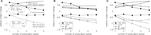Figure 14. Presynaptic burst pairing protocol (5), full lines: pre-burst-post case, experiments and reproduction, dashed lines post-pre-burst case, experiments and reproduction. (A) standard STDP, (B) LCP with LIAF with nearest-neighbor interaction, (C) LCP with LIAF with all-to-all interaction. For the Θu curve in (B) and (C), we set Θu = 1 mV; for the Θu + PSP curve, we set Θu = 2 mV and UPSP = 1 mV; for the “none” curve, we set Θu = 0 mV and UPSP = 0 mV. STDP parameters taken from Froemke et al. (2006) (cf. Froemke 2 parameter set, see also discussion at Figure 3 for LCP with SRM in Table 4, additional LCP with LIAF parameters (interaction mode, UPSP) as stated in the last sentences).

Figures 14B,C shows that LCP with LIAF has similar problems in reproducing the experimental results. Without extensions, LCP with LIAF behaves essentially like standard STDP. Because the protocol employs only one postsynaptic spike, behavioral differences due to the resetting of the postsynaptic membrane potential do not appear; also, attenuation of postsynaptic spikes is effectless. All other parameters affect both pre-post and post-pre pairings in the same direction. Increasing the voltage threshold Θu adds depression that increases with the number of presynaptic spikes, because the (constant) negative voltage difference (u − Θu) is integrated over a longer time, multiplied with more presynaptic activity. Postsynaptic potentials counterbalance this effect, because they raise the membrane potential. Replacing nearest-neighbor with all-to-all interaction strengthens the influence of postsynaptic potentials and otherwise amplifies the weight change with increased number of presynaptic spikes, because presynaptic activity is accumulating. Incorporating all these effects, either pre-post or post-pre behavior can be reproduced, but not both with the same parameter set. Like for the standard STDP rule, the difference between the weight changes for the pre-post and the post-pre case increases in both versions of the LCP rule, but it decreases in the experimental results.

## Discussion

From the experiments described in Artola et al. (1990), Holthoff et al. (2006), Kampa et al. (2007), Lisman and Spruston (2005), Ngezahayo et al. (2000), and Sjöström et al. (2008), it can be postulated that a significant ingredient of synaptic plasticity are localized, voltage driven processes. We have taken this hypothesis one step further, creating a plasticity rule where the complete synaptic plasticity is dependent on the postsynaptic membrane potential. Is this supported by the mechanisms underlying the generation of synaptic plasticity? According to (Aihara et al., 2007; Sjöström et al., 2008), a slow inactivation of the Calcium channels following a medium Calcium elevation is necessary for LTD, whereas a fast Calcium spike produces LTP. According to the time constants and voltage dependencies shown on page 217 of Koch (1999), a membrane potential below resting (<−65 mV) produces this slow inactivation. If a PSC arrives during the refractoriness period, it would lead to a temporary increase in membrane potential (while staying below resting potential), thus producing this medium Calcium activation followed by a prolonged inactivation. This mechanism is replicated in the LCP rule by the convolution of the PSC with the membrane potential during the refractoriness period. However, if the membrane potential is above rest when the PSC arrives, the shorter time constant governing this operating region of the membrane voltage (see Figure 9.3 of Koch, 1999) produces a sharp increase and subsequent decay in Calcium levels as a response to the PSC, thus forming LTP (Aihara et al., 2007). In our LCP rule, the membrane potential is above rest for a postsynaptic action potential and (for LCP with LIAF) for PSC-caused sub-threshold elevations of the membrane. When this depolarized membrane potential is convolved with a coincident PSC, the rule produces LTP.

The LCP rule is thus driven by the underlying short term dynamics of the neuron and synapse. The mechanisms producing LTP and LTD are similar to (Saudargiene et al., 2004; Shah et al., 2006), with the weight determined by a convolution of pre- and postsynaptic waveforms. Introducing a voltage dependence for such a rule, however, does not necessarily result in a plasticity function dependent on voltage. For example, in Saudargiene et al. (2004), the voltage dependence is cancelled out by the symmetric scaling of both the LTP and LTD part of the STDP curve. Similarly, the model of Saudargiene et al. (2004) indicates that a voltage-dependent threshold does not necessarily translate in a BCM-like frequency threshold. In contrast, we show in Eq. 23 for LCP with SRM a direct relation between the sliding frequency threshold as defined in the BCM rule and the membrane potential threshold of Eq. 2. Also, we show the direct plasticity-voltage dependence as defined in Eq. 2 for the experiment in Figure 12C, albeit only with the LTP/LTD threshold emulated.

The LCP rule, while simple in itself, can replicate complex behavior by incorporating a realistic synaptic/dendritic environment. For example, the inclusion of the AP attenuation (Figure 7) or the addition of the PSC influence on the postsynaptic membrane potential (see LCP with LIAF Neuron) resulted in a clear improvement in the ability of the model to replicate experimental findings. These examples show that the LCP rule is very amenable to combinations with further presynaptic or postsynaptic adaption mechanisms (Farajidavar et al., 2008). For example, we expect that a presynaptic adaptation such as the one described in Markram et al. (1998) would resolve the discrepancy of the LCP rule with respect to presynaptic bursts (5). This would be similar to what Froemke et al. (2006) achieved when making their revised suppression model dependent on all presynaptic pulses.

Of course, when including additional effects in a plasticity model, there is always the risk of simply fitting the model to specific experimental data. For example, while the state-machine model of Lu et al. (2007) might actually capture some underlying biophysical mechanisms, the fact that its parameters have to be fitted individually for each experiment argues against this. Another example would be the model of Clopath et al. (2010), which extends the original triplet model of Pfister and Gerstner (2006) with two voltage thresholds to replicate the data of Artola et al. (1990) and Ngezahayo et al. (2000). While this extension works well, the model produces significantly shorter time constants than the original triplet model after being fitted for experimental data. Thus, an additional longer time constant seems to have been introduced through the depolarizing after-potential of the neuron so that the plasticity model would replicate realistic STDP time windows.

In this context, we argue that the inclusion of the postsynaptic adaptation in both versions of the LCP rule is not simply a fit to experiments. Rather, it is in broad agreement with actual experimental evidence and mechanisms. For example, similar to our implementation, this adaptation chiefly alters the size (amplitude and duration) of the postsynaptic action potential (Tanaka et al., 1991; Froemke et al., 2006), and the adaptation has the postulated effect on plasticity (Froemke et al., 2006). Also, the time constant is compatible (Shah et al., 2006). The deflection of the postsynaptic membrane voltage due to the charge represented by the PSC (as included in LCP with LIAF) can, of course, also be motivated from experimental evidence (Artola et al., 1990; Koch, 1999). The veracity of including presynaptic adaptation similar to (Markram et al., 1998) in models of plasticity is proven experimentally in Froemke et al. (2006). We did not include a mechanism for weight dependency in the LCP rule, i.e., all weight changes that are sufficiently separated in time sum linearly. This is because the experimental evidence and computational effect of weight-dependent plasticity is still a matter of controversy (Standage and Trappenberg, 2007; Morrison et al., 2008). Furthermore, the weight change would depend on the initial weight, a parameter that is unknown in most experimental setups. In the LCP rule, a weight dependence would most naturally be included in the conductance g(t), leading to equal weight dependence for LTP and LTD.

Regarding the actual weight computation in the LCP rule, a continuously generated synaptic weight based on local state variables seems more in line with the also continuously operating biological processes at the synapse than the assumption of purely discrete, spike timing-driven events as in STDP-type models. For example, a single exponential relating spike time to weight change in classical STDP rules seems an artificial simplification when compared to the spread of results for actual experiments (Bi and Poo, 1998). Other plasticity rules take a fundamentally stochastic approach to modeling synaptic plasticity (Elliott, 2008), which also replicates the variation seen in classical STDP experiments. However, those rules cannot reconcile the stochastic nature of spike timing experiments with the more deterministic behavior for voltage control (Ngezahayo et al., 2000; Sjöström et al., 2001) or dendritic spike experiments (Holthoff et al., 2006; Kampa et al., 2007). In the LCP rule, deterministic or stochastic deflections of the membrane voltage could account for a significant proportion of the statistical spread (see the sweeps in Figures 5A and 13B). Even for controlled postsynaptic conditions in plasticity experiments, such as APs induced by current pulses or even for a voltage clamp of the soma, the local dendritic membrane potential is subject to stochastic variation (Artola et al., 1990) and thus to these possible influences on plasticity.

To further a general model comparison, a detailed discussion of a broad selection of plasticity models is included in the Appendix, with a summarized comparison derived from this discussion given in Table 3. The discussion also includes estimated or proven performance of a model against a fixed set of eight experiments described in Table 1. In the original publications corresponding to each model, these are only verified against an arbitrary sample set of experimental data (usually significantly smaller than the listing provided in Table 1), making a performance comparison of the models very difficult. In contrast, we attempt to describe a “set union” of experiments which incorporates significantly differing aspects of spike-, rate-, and voltage-based plasticity, requiring a model to contain mechanisms that replicate these differing aspects. Single mechanisms can then be extracted from Table 3 which exhibit both good experiment reproduction and are common to several models. These mechanisms may in turn hint at some underlying biophysical process.

For example, based on the comparison, a case can be made for plasticity influenced by an exponential form of postsynaptic adaptation (Badoual et al., 2006; Froemke et al., 2006; Pfister and Gerstner, 2006; Shah et al., 2006), which is included in the LCP rule. LCP also exhibits plasticity dependent on waveforms of local state variables (Shouval et al., 2002; Saudargiene et al., 2004; Badoual et al., 2006; Shah et al., 2006). As suggested by computational analysis (Pfister et al., 2006), the LTP time constant of the LCP rule is equivalent to the PSC time constant, while LTD is based on the membrane time constant. Voltage control is realized in the form of a threshold applied to the postsynaptic membrane potential similar to the models of Clopath et al. (2010), Fusi et al. (2000), and Sjöström et al. (2001). Reproduction of rate behavior (Dudek and Bear, 1992) in the LCP rule is assured by applying an event-based form of the pre- and postsynaptic activity to a BCM-like rule as in Toyoizumi et al. (2005). Spike timing is inherent in the LCP rule in the form of starting points of PSCs, APs and the refractoriness period similar to (Abarbanel et al., 2002; Badoual et al., 2006), but not explicitly modeled as discrete events such as in Froemke et al. (2006), Izhikevich and Desai (2003), and Pfister and Gerstner (2006). Compared to kinetic or state transition models (Abarbanel et al., 2002; Senn, 2002; Lu et al., 2007), which generate a waveform based on kinetic mechanisms and then compute the resultant weight change based on this waveform, the LCP rule omits this step, taking the (idealized) waveform directly as input. The actual weight generation in the LCP rule based on these waveforms is somewhat similar to trace-based versions of spike timing-driven plasticity rules (Senn, 2002; Badoual et al., 2006). However, the traces are not merely sampled at the respective opposite event (presynaptic trace for postsynaptic event and vice versa), rather they are convolved and integrated across time. This convolution with a time-continuous presynaptic waveform is also the main difference to the model of Fusi et al. (2000).

Overall, the LCP rule provides a very compact implementation of the above mechanisms. As seen in Table 3, both LCP with SRM and LCP with LIAF combine a very general experiment reproduction ability with one of the lowest parameter counts. Legitimately, the LCP rule could even be interpreted as containing only the voltage threshold and one weight scaling constant as in Eq. 2, since all the behavior shown in this paper can be replicated with a reasonable model for the synaptic current and neuron, which are already contained in most simulations or hardware realizations (Indiveri et al., 2006; Schemmel et al., 2007). Our LCP rule would then be reduced to Eq. 2, while the time functions of PSC and postsynaptic membrane potential are taken from other parts of the system. The downside of such an approach would be the reduction in flexibility of the learning rule, making it very dependent on the details of the postsynaptic modeling. However, it significantly reduces the computational effort involved in implementing this rule in hardware or software compared to other STDP/BCM formulations (Mayr et al., 2010a).

The parameters of the LCP rule may also be metaplastically adjusted in a fashion similar to Figure 5 to replicate metaplasticity mechanisms experimentally observed in vivo (Lebel et al., 2001; Zelcer et al., 2006). For example, reduced post-spike after-hyperpolarization (AHP) seems to be related to enhanced learning in experimental animals (Disterhoft and Oh, 2006; Zelcer et al., 2006). In the LCP rule, this would correspond to reduced spike attenuation or reduced refractoriness and thus a predisposition toward LTP (see Figure 5C). However, after the training is concluded, not only is the AHP again reduced to a relaxed state (Disterhoft and Oh, 2006), thus balancing LTD and LTP, there is also an overshoot toward enhanced LTD which can be observed in experimental animals (Lebel et al., 2001). Thus, a second effect such as an increase of Θu in the LCP rule might follow this reduced AHP and outlast it once the AHP has again assumed a naive state. Do the corresponding time constants support this course of events? From Lebel et al. (2001) and Zelcer et al. (2006), the time constant for changes in AHP can be inferred as approximately 1 day. If we assume Θu to be changed by voltage-based metaplasticity similar to (Ngezahayo et al., 2000), there seems to be a contradiction, since this metaplasticity is induced more rapidly than the AHP change and would probably also decay long before the respective AHP change, not outlast it. However, this may not represent the true time constant inherent in this form of metaplasticity. We have already established a link between the voltage and frequency thresholds (see Eq. 23), so in this context, we may assume the voltage-based (Ngezahayo et al., 2000) and rate-based (Wang and Wagner, 1999; Abraham et al., 2001) metaplastic effects to be caused by a similar mechanism. Both can be established very rapidly by strong stimuli (Wang and Wagner, 1999; Ngezahayo et al., 2000). This is similar to the one-shot plasticity induction of Holthoff et al. (2006), which does, however, not represent the actual time course of plasticity induction in vivo (Lisman and Spruston, 2005). In the same vain, the actual time constant of the metaplastic LTD/LTP threshold could rather be inferred from its relaxation time, i.e., the time it takes to return to a naive state, which is about ten days (Abraham et al., 2001). This would support the view above, i.e., that the AHP reduction is first activated by a learning task, followed by a slower metaplastic or homeostatic change in the LTD/LTP threshold, which outlasts it after the training is over. In the LCP rule, these AHP changes could be emulated through the adjustment of the extension τrefr and depth Urefr of the refractoriness. At the same time, a second slower process would adjust Θu (see Figure 5) to account for the change in LTP/LTD threshold.

Thus, at the price of two additional metaplasticity time constants and the corresponding equations providing long-term adjustments of τrefr and Θu, the LCP rule could be extended to provide a holistic yet parsimonious method for exploring the so far little model-accessible field of metaplasticity, while at the same time retaining the ability to replicate a wide range of conventional experimental plasticity data as shown in this manuscript.

## Appendix

### Review of Plasticity Models

In the following, each paragraph is devoted to a short discussion of a current plasticity model, which, together with the classification of plasticity mechanisms of Figure 2 and the motivational classification of Figure 1, form the basis for the model comparison in Table 3. Where possible, Figure and manuscript references for a given model and experiment are detailed in the text.

#### Conventional STDP

(Song et al., 2000; Izhikevich and Desai, 2003): Since the conventional exponential formulation of STDP has originally been proposed to reproduce STDP (1) of Table 1, this experiment poses no problem (Figure 1E in Froemke and Dan, 2002), receiving classification “f” in Table 3. Experiments (2)–(5) rely mainly on some type of short time adaptation, which is not included in basic STDP. The unsatisfactory behavior of conventional STDP with respect to experiments (2), (4), (5) is shown in Figures 10A, 8B, and 14A, thus receiving “n.” Triplets (3) are partially reproduced by STDP (Figure 7D), the short time adaptation being only necessary for one quadrant, thus STDP is classified as “h” with respect to this experiment. Conventional STDP is not able to reproduce standard rate (6) (Figure 11C). STDP is able to reproduce BCM behavior for a non-stochastic presynaptic rate sweep as in experiment (6), if the postsynaptic spike is assumed to occur exactly in the middle between two presynaptic spikes (Standage et al., 2007). However, since this would assume a very specific rate-dependent pre-post latency which is likely incompatible with actual neuron behavior, we do not take this reproduction into account in Table 1. Also, this STDP protocol is not able to reproduce correlated rate (7), as argued in Section “Benchmark Experiments” (see also Figure 6A).

However, the performance of STDP with respect to the rate experiments (6) and (7) is said to depend on the exact implementation of the spike interaction (Izhikevich and Desai, 2003). The STDP modification of Izhikevich and Desai (2003) employs a nearest-neighbor protocol that is centered on the postsynaptic side, i.e., a presynaptic pulse is combined with the immediately preceding and subsequent postsynaptic pulses. It is claimed that this modification makes conventional STDP compatible with BCM. However, at least for the assumption of a fixed postsynaptic Poisson spiking, the presynaptic rate sweep of standard rate (6) has no discernible influence on the plasticity curve (Figure 3 in Mayr et al., 2010b), producing a flat line that can be altered only through the postsynaptic side. This is of course somewhat due to the postsynaptic stimulus assumption, since the STDP protocol of Izhikevich and Desai (2003) is centered around a postsynaptic sweep, not a fixed rate. However, Standage et al. (2007) have shown that STDP cannot reproduce BCM behavior for several other possible postsynaptic reconstructions of Dudek and Bear (1992) even when the protocol of Izhikevich and Desai (2003) is applied.

Also, the spike protocol assumption of Izhikevich and Desai (2003) is somewhat artificial, since conventional rate experiments are stimulated with a changing rate on the presynaptic side, not a fixed one. Even with changing presynaptic rate, this experimental protocol is very unlikely to lead to a postsynaptic rate that experiences a frequency sweep, but has single spikes totally uncorrelated to the presynaptic spike times, as is assumed for the postsynaptic Poisson sweep in that publication. A more natural assumption would be a strong correlation between presynaptic and postsynaptic rate, with a weak correlation between individual pre- and postsynaptic spike times, as expressed in the stimulation protocol of correlated rate (7). In the context of this experiment, the STDP modification of Izhikevich and Desai (2003) is unable to reproduce experimental results (Figure 2 in Mayr et al., 2010b). Again, the STDP curve is sampled on either side of the postsynaptic pulse, leading to LTP behavior for realistic STDP parameters (i.e., with LTP dominating LTD at short time intervals). Since there is no real difference in simulation results, the more widely used STDP protocol of Morrison et al. (2008) (nearest-neighbor) is employed for STDP simulations. Voltage Control (8) necessitates a mechanism relating voltage to plasticity, which is not included in STDP.

#### Froemke et al. (2006)

The revised suppression model of Froemke et al. (2006) is an extension of the conventional, pair-based STDP formulation. The spike efficacies A+ and A of STDP are governed by time-averaged versions of the pre- and post-spike train, where short inter-spike-intervals (ISI) have a depressing effect, i.e., the influence of the corresponding pre-post-spike pair on overall plasticity is diminished. The revised suppression model is an extension of the original suppression model (Froemke and Dan, 2002), where A+ and A scale with the complete history of the presynaptic spike train rather than just the last ISI. Standard STDP behavior is not explicitly shown for the revised suppression model, but can very likely be reproduced since both the original and revised suppression model reduce to STDP for low pairing frequencies. An experiment similar to frequency-dependent STDP (2) of Table 1 is shown to be valid for the model, but this experiment only covers negative pairing time differences. The reproduction of the behavior in experiment (2) for positive time differences (pre-post pairings), is not possible (Figure 15). There is very high LTP at low pairing frequencies, not the zero weight potentiation found in Sjöström et al. (2001), caused by the essentially STDP nature of the model at these frequencies. For an increase in pairing frequency, the LTP decreases steeply to the amplitude difference between LTP and LTD bounds (the horizontal line between 15 and 70 Hz), since a very large amount of LTP is still being generated by the pre-post pairings, but there is an increase in postsynaptic relative to presynaptic efficacy suppression, due to the differing time constants. The effect of the later postsynaptic spikes diminishes, causing a large increase in LTD. For frequencies above 70 Hz, the presynaptic spikes in each burst are also suppressed, so that the equivalent protocol would reduce to conventional one-pairing STDP (all spikes after the first spike in each burst are suppressed), leading to enhanced LTP. Turning off the weight bounds of Froemke et al. (2006) completes the curve now obscured by the horizontal line, but results in no qualitative difference.

FIGURE 15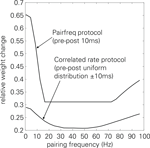Figure 15. Reproduction of frequency-dependent STDP (pre-post pairings) and of the correlated rate protocol for the revised suppression model of Froemke et al. (2006), parameters from Froemke et al. (2006).

The original suppression model is able to reproduce triplet (3) behavior (Figure 3B in Froemke and Dan, 2002). However, triplet behavior for the revised model discussed here has only been shown for the limited number of cases which overlap with the burst experiments of Froemke et al. (2006). It is somewhat likely that the revised suppression model is also compatible with the other triplet experiments, since the newly introduced dependence on all presynaptic pulses reduces to the immediately preceding one for low triplet repetition frequencies. However, it is not clear what consequence the postsynaptic scaling and the significantly longer postsynaptic efficacy time constant have especially in the post-pre-post case. The reproduction of quadruplet (4) behavior is not shown, but is also likely since quadruplets are replicated with the original suppression model (Figure 3C in Froemke and Dan, 2002) and also because of the similarities between (Froemke et al., 2006) and the model of Pfister and Gerstner (2006). The revised suppression model is of course able to reproduce burst (5) plasticity (Figures 4B,D in Froemke et al., 2006), since it is built on the basis of those experiments.

As derived analytically by (Izhikevich and Desai, 2003), the original suppression model of Froemke and Dan (2002) is not able to reproduce BCM-like behavior for Poisson spike trains. This is also evident from the simulations documented in Figure 3 of Froemke et al. (2006), where the original suppression model fails to cross from LTD to LTP with rising burst frequency. However, the same diagram shows BCM-like behavior for the revised suppression model. Thus, the nearest-neighbor protocol of Izhikevich and Desai (2003) is not the only way to make STDP compatible with protocols showing BCM-like behavior, the presynaptic all-to-all suppression of spike efficacy in the revised suppression model (Froemke et al., 2006) can evidently serve the same purpose.

However, the model is still incompatible both with the standard rate experiment (6) for all postsynaptic settings (Figure 3 in Mayr et al., 2010b) and with correlated rate (7). Replicating the correlated rate experiment in the revised suppression model with the parameter set of Froemke et al. (2006) leads only to LTP behavior, with 30% weight increase for low pairing frequencies (Figure 15). As argued above for STDP in connection with this experimental protocol, this is caused by the sampling/summation of the asymmetric STDP LTP/LTD amplitudes. The overall curve progression to higher pairing frequencies is caused by similar effects as cited above for the pre-post frequency-dependent STDP protocol, with a decrease in LTP and subsequent increase at very high pairing frequencies. However, the amplitude is reduced due to the averaging/scaling effect of having both post-pre and pre-post pairings at varying time differences. There is no mechanism for voltage control (8) included in the model.

#### Pfister and Gerstner (2006)

The model of Pfister and Gerstner (2006) is another extension of the conventional, pair-based STDP formulation. Additional to the presynaptic and postsynaptic pulse traces used in iterative implementations of standard STDP (Morrison et al., 2008), secondary presynaptic and postsynaptic trace variables with separate time constants are introduced. These new traces modulate the weight change: at a postsynaptic spike, the weight is increased dependent on the first presynaptic trace like in standard STDP, but the amplitude of the LTP weight change is increased with the second postsynaptic trace. Likewise, the LTD amplitude is modulated by the second presynaptic trace. Thus, these new traces introduce a form of adaptation to the presynaptic and postsynaptic side. However, in contrast to the model of Froemke et al. (2006), previous spiking activity increases LTD and LTP amplitude. Pfister and Gerstner (2006) show that the second presynaptic trace in their model can be neglected without significantly impairing reproduction of experimental results, reducing the model to 3 time constants and 3 scaling factors. We therefore use this simplified model in our comparison. Standard STDP (1) (Figure 3 in Pfister and Gerstner, 2006) and quadruplet (4) (Figure 5B in Pfister and Gerstner, 2006) protocols pose no problem to the model. Furthermore, the model can well reproduce frequency-dependent STDP (2) (Figure 5A in Pfister and Gerstner, 2006). Regarding experiment (3), the model of Pfister and Gerstner (2006) is built to replicate the triplets of Wang et al. (2005) (Figures 5C,D in Pfister and Gerstner, 2006), not the ones of Froemke and Dan (2002). The presynaptic burst pairing protocol (5) can very likely not be accounted for by the reduced Pfister and Gerstner (2006) model, because it does not incorporate presynaptic adaptation and thus performs like STDP. Even the full model is likely to be not compatible with the experimental results, because for the pre-burst-post protocol, its presynaptic adaption is not included in the weight change and for the post-pre-burst protocol, presynaptic adaptation leads to increased LTD, conflicting with the measurements. The authors show an analytical relationship to BCM behavior for their rule for presynaptic and postsynaptic Poisson spike trains. However, this derivation cannot be generalized to experimental protocols as discussed in Section “Benchmark Experiments,” e.g., standard rate (6) is not reproduced (Figure 3 in Mayr et al., 2010b). In the nearest-neighbor version, the reduced model is compatible with correlated rate (7) (Figure 2B in Mayr et al., 2010b). Voltage control experiments (8) can not be reproduced by the model, because it does not include a dependency on membrane potential.

#### Benuskova and Abraham (2007)

The STDP modification described in Benuskova and Abraham (2007) aims to provide a level of metaplasticity to the standard STDP formulation. The positive and negative weight scaling factors are adjusted by dividing respectively multiplying with the mean of the postsynaptic pulse activity across a 60 s time window. The expression used for the sliding mean is somewhat problematic in the context of the BCM formulation of Izhikevich and Desai (2003). Benuskova and Abraham (2007) state that their ΘM translates in a sliding of the frequency threshold ϑ computed in Izhikevich and Desai (2003). However, when computing the original ϑ of Izhikevich and Desai (2003) as a function of the weight scaling “threshold” ΘM for the STDP parameters used in Benuskova and Abraham (2007), only a very narrow useful range of ΘM can be found where the resulting ϑ forms a valid threshold. This range starts at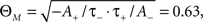where ϑ crosses to positive values, and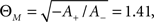where ϑ has a pole, i.e., the threshold jumps to infinity. In reality, this range is even smaller, since ϑ leaves the range of useful frequency values at about ΘM = 1.2. At ΘM values above the pole, ϑ is negative, reaching ϑinf = −1/τ+ when ΘM goes to infinity. Since this dynamic range for ΘM is quite narrow, a ΘM that according to Eq. 10 of Benuskova and Abraham (2007) varies almost linearly with the pulse frequency (i.e., exhibits the same dynamic range) is not compatible with the declared goal of the authors of providing ϑ of Izhikevich and Desai (2003) with a valid metaplastic variation ability. The scaling constant α given in Benuskova and Abraham (2007) would also result in ΘM >> 1.41 and thus in a negative frequency threshold. As to the experiment reproduction, the model of Benuskova and Abraham (2007) can probably show the conventional STDP (1) behavior it is originally derived from, although the metaplasticity time constant (60 s) is longer than the usual pairing separation distance in experiments (10 s), which might result in a change in the shape of the STDP curves during the course of the experiment. The assessment for experiments (2)–(5) and (7) is as stated above for conventional STDP, the metaplasticity time constant is too large to significantly affect the models’ behavior with respect to these experiments. Although a protocol similar to (Dudek and Bear, 1992) is employed in eliciting heterosynaptic metaplasticity, standard rate (6) behavior is not demonstrated, i.e., no frequency sweep is carried out (Figure 1 in Benuskova and Abraham, 2007). Also, the link between the sliding threshold and the postsynaptic membrane potential is not clear, so the derivation cannot be used to extend STDP to voltage control (8).

#### Senn (2002)

This paper models the discharge probability Pdis of a synapse for each incoming pulse as being modified in a plastic way, equivalent to the synaptic strength/weight in other plasticity mechanisms. A set of receptors N is introduced that can make transitions from a recovered state Nrec either into an upregulating state Nup triggered by a presynaptic spike, or into a downregulating state triggered by a postsynaptic spike Ndn. Two secondary messengers Sup and Sdn are used to generate a time-averaged version of these receptor states. For generation of LTP, the running mean of the presynaptic activity as stored in its corresponding secondary messenger is weighted with a threshold and then read out when a postsynaptic pulse occurs, which upregulates Pdis. The opposite mechanism (presynaptic spike samples mean of postsynaptic activity) is active for the downregulation (LTD). This is similar to a trace-based version of STDP (Morrison et al., 2008), with additional pre- and postsynaptic adaptation through the secondary messengers. For low frequency pairings as in the conventional STDP (1) protocol, very little overall plasticity is produced (Figure 6A in Senn et al., 2001). For higher pairing frequencies, the curve shape diverges significantly from the conventional exponential STDP assumption, but still seems a good reproduction (Figure 6B in Senn et al., 2001). With regard to frequency-dependent STDP (2), only the pre-post pairing sweep is reproduced (Figure 1B in Senn, 2002). Due to the threshold property of the model, post-pre pairings start at zero potentiation for low frequencies, not at significant LTD as seen in the experimental data. Triplets (3) are reproduced by the model (Figure 2A in Senn, 2002). Quadruplet (4) reproduction is somewhat likely, since there exists both pre- and postsynaptic attenuation in the model, but has not been shown. Burst (5) is expected to be compatible with the model, since presynaptic adaptation/depression is part of the model and since at least an increasing number of pairings shows decreasing LTP (Figure 5A in Senn et al., 2001). An experiment similar to standard rate (6) is shown to be compatible with the model (Figure 1D in Senn, 2002). Correlated rate (7) can likely be reproduced, since at least the pre-post case of frequency-dependent STDP is compatible with the model. As to voltage control (8), there are two separate thresholds for LTP and LTD included in the model, but their mapping to voltage remains unclear.

#### Abarbanel et al. (2002)

This model is based on kinetic equations, i.e., first order differential equations which govern the transition between two states of a system. The processes represented by the differential equations are presynaptic neurotransmitter release P(t) and postsynaptic activity D(t), with disturbance functions that represent pre- and postsynaptic action potentials. Both processes are cross-wise added to derive the weight in a mixture of competition and cooperation, i.e., both are needed for a weight change, but the relative timing of events in P(t) and D(t) determines the sign of the weight change. Pre- and postsynaptic competition respectively cooperation as a source for LTP and LTD has also been shown experimentally (Tzounopoulos et al., 2007). Based on the fact that the kinetic equations for dirac-like disturbance functions can be solved as exponential functions scaled with the dirac area, expressions for STDP-like single-spike pairings as well as random spike trains are derived. Based on the STDP (1) plasticity replication shown in these analyses (Figure 1 in Abarbanel et al., 2002), a parameter fit to STDP is carried out which is used for simulating other spike protocols. Frequency-dependent STDP (2) is replicated qualitatively (Figure 2 in Abarbanel et al., 2002, with LTP/LTD behavior at low pairing frequency, the difference between both curves decreases with increasing frequency, the LTP curve increases, the LTD curve crosses over to LTP). However, there are significant differences in the quantitative reproduction, e.g., relative to the LTP value of both curves for 50 Hz, both start out at almost zero weight change, i.e., the LTD behavior of the low frequency pairings and the initial difference between both curves is almost insignificant. As evident from this experiment, the model offers pre- and postsynaptic superposition, i.e., the effects of subsequent pulses overlap. However, no pre- or postsynaptic adaptation is included where earlier pulses influence subsequent ones. Thus, Triplets (3), which rely on postsynaptic adaptation, can probably be reproduced by the model only in an STDP-fashion. Likewise, bursts (5) rely on presynaptic adaptation, and quadruplets (4) on pre- and postsynaptic adaptation, which likely make them incompatible with the model of Abarbanel et al. (2002). As to standard (6) and correlated (7) rate, although BCM-like behavior could be inferred from the reproduction of experiment (2), a standard pre- and postsynaptic Poisson sweep produces only LTP (Figure 3 in Abarbanel et al., 2002). If the clamped postsynaptic behavior in Figure 5 of Abarbanel et al. (2002) is taken to represent membrane voltage, the model of Abarbanel et al. (2002) seems to provide at least an LTD/LTP threshold, so voltage control (8) can probably be partially replicated.

In this model, biophysically realistic formulations for a compartmental neuron and an AMPA/NMDA synapse are given, and a two-state kinetic plasticity process is assumed, with separate artificial LTP and LTD “enzymes.” The behavior of the model is similar to other kinetic models (Abarbanel et al., 2002; Lu et al., 2007; Zou and Destexhe, 2007), producing a conventional STDP (1) curve with a soft crossover from LTP to LTD at small time differences between pre- and postsynaptic pulses (Figure 6 in Badoual et al., 2006). The plasticity derivation is similar to (Saudargiene et al., 2004), i.e., since the weight update is a function of the underlying waveforms (e.g., Calcium), the STDP weight change curve is a function of the waveform characteristics (time constants, amplitudes). Similar to STDP, the biophysical model partially reproduces triplet (3) behavior (Figure 11C in Badoual et al., 2006, compare also Figure 7D). Frequency-dependent STDP (2) is reproduced by this model, albeit with significantly lower LTD/LTP threshold frequency (Figure 7A in Badoual et al., 2006). Quadruplets (4) are not tested for the model, but its performance is probably similar to STDP, based on the triplet results. The performance with respect to the experiments (5), (6), and (7) involving rate changes is unknown, since the model on the one hand performs very similar to STDP for some experiments, but also incorporates rate effects which enable it to reproduce experiment (2). With regard to voltage control (8), there exist possibly voltage-dependent state variables (e.g., Ca2+ concentration), but it is not clear if those state variables can be influenced by a voltage signal in a way that would produce behavior compatible with experiment (8).

In a second modeling approach, the authors carry out a simplification of their biophysical model, essentially reducing it to conventional STDP, then add pre- and postsynaptic efficacy traces (for current and preceding spike). Basically, this results in a copy of the original suppression model of Froemke and Dan (2002), with added soft weight bounds, but as stated in Badoual et al. (2006), these bounds do not affect its basic behavior. STDP behavior (1) is replicated (Figure 4B in Badoual et al., 2006), while frequency-dependent STDP (2), cannot be replicated (Figure 7B in Badoual et al., 2006). Triplets (3) are naturally fully compatible with the model (Figure 11D in Badoual et al., 2006, also Figure 2A in Froemke and Dan, 2002). Quadruplets and presynaptic bursts (5) are fully replicated (Figure 3C in Froemke and Dan (2002) respectively Figures 4B,D in Froemke et al., 2006). The performance with respect to the rate experiments (6) and (7) is very likely similar to the model of Froemke et al. (2006) without weight bounds, which would still leave the model incapable of replicating the experiment (Figures 2 and 3 in Mayr et al., 2010b). A voltage threshold/dependence (8) is not included in the model. A slightly different version of this model is introduced in Zou and Destexhe (2007), with the soft weight bounds and the pre- and postsynaptic efficacy traces directly coupled with the kinetic model. Since this only introduces the same spike efficacies as the original suppression model (Froemke and Dan, 2002) onto a basically STDP curve shape, the results with regard to experiments (1) through (8) should be the same as those discussed for the simplified model of Badoual et al. (2006).

#### Lu et al. (2007)

This paper presents a finite state model with a resting, a pre and a post state governed by the local actions at the synapse. Transitions between states are caused by a change from a pre- to a postsynaptic pulse (and vice versa), as well as between two consecutive pre- or postsynaptic pulses. This is somewhat similar to the transitions between states in a kinetic model. Each transition starts a decaying and an increasing exponential trace with different time constants, with the amplitude of the exponential trace read-out at the time difference between the pulses defining the transition (pre-pre, pre-post, post-pre, post-post). Both traces are multiplied to form a weight change rate. The weight change rates for all transitions are then non-linearly weighted in a weight-saturation (soft bound) scheme and added to arrive at the overall weight change. A drawback of this model is that it employs the same time constants and scaling factors for pre- and postsynaptic adaptation as for the pre-post and post-pre weight derivation function. Consequently, while the model is able to replicate a range of experimental results, a new parameter set (with values differing by a factor of 10) is required for each new experiment, even though all experimental data is derived from very similar synapses (same preparation, brain region, animal, etc.), and should therefore be reproducible with a single parameter set. Experiments (1), (3), and (4) are replicated (Figure 2 in Lu et al., 2007; Table 1 in Lu et al., 2007; Figure 3 in Lu et al., 2007,respectively). The error fit for quadruplets (4) results in a different, but still faithful approximation curve compared to the usual assumption in Hartley et al. (2006) and Pfister and Gerstner (2006). With regard to any of the experiments involving rate changes, an analytical expression similar to (Appleby and Elliot, 2005) for a pre- and postsynaptic Poisson sweep is developed, which shows typical BCM behavior. However, the derivation of Appleby and Elliot (2005) relies on rate-dependent changes in the distribution of the pre-post time difference, which would make this derivation (and possibly the entire rule) incompatible with correlated rate (7). The model may be able to reproduce standard rate (6) at least for some postsynaptic reconstructions, since this experiment is more in line with the Poisson sweep of the mathematical derivation in Appleby and Elliot (2005) and Lu et al. (2007). Since it is not proven whether the model is compatible with experiments involving a fixed pre-post distribution in combination with a frequency sweep, the reproduction of frequency-dependent STDP (2) is also unclear. Bursts (5) are probably compatible since they involve a presynaptic adaptation also required for the conventional triplet experiment (compare burst reproduction with original triplet model in Figures 4B,D in Froemke et al., 2006). Regarding experiment (8), there is no mechanism for voltage control; the model is purely spike timing governed.

#### Pfister et al. (2006)

A learning rule is derived which lets a postsynaptic neuron fire at precise moments in time relative to some presynaptic input. The presynaptic input is modeled similar to the PSC of Eq. 3, but with a second exponential producing an additional rise time. The neuron is also similar to Section “Neuron Dynamics: Spike Response Model,” i.e., a simple spike response model as in Eq. 5, but without the action potential and with escape noise added to the firing threshold. Based on this neuron and a supervised learning paradigm, the neuron has to learn to spike relative to presynaptic single spikes, a spike ensemble and a spike pattern (repeated spikes by the same neuron relative, combined over a population). Constraints are used in the form of a penalty for spiking at undesired times, or a mean target firing rate, or in restricting the learning rule to temporal locality. Extracting the equivalent STDP window from the learning rule, the LTP for pre-post pairings is always governed by the time constant of the presynaptic pulse, reflecting the charging of the membrane capacitance by the EPSP. LTD for post-pre pairings results from the learning rule only if constraints are used. In most cases, the LTD side reflects the refractoriness characteristics of the spike response model (time constant and amplitude). Thus, STDP behavior (1) is shown (Figure 3B in Pfister et al., 2006). Since no adaptation or general second order effects are integrated in the model and its plasticity curve reflects STDP, the performance with respect to the other experiments is expected to be very similar to conventional STDP (Song et al., 2000). The reproduction of the rate experiments (6, 7) is probably similar to (Baras and Meir, 2007), i.e., such supervised optimized learning rules can replicate BCM in a fashion similar to (Izhikevich and Desai, 2003), with its attendant shortcomings as discussed for STDP. Computational learning rules based on neuron characteristics such as refractoriness can be made more broadly compatible with BCM terminology by incorporating additional rate-dependent state variables (Toyoizumi et al., 2005). However, the model of Pfister et al. (2006) lacks these higher-order dependencies. Computational models can also be extended toward biophysically realistic formulations. For example, Saudargiene et al. (2004) introduce a biology-driven plasticity function that develops the computational capability of detecting correlations between input and output signals in an unsupervised scenario. Similar to (Pfister et al., 2006), the LTP part of the STDP window is based on the shape of the EPSP. However, the LTD for the post-pre case is dependent on bAP, not on refractoriness characteristics. Different forms of STDP are accounted for by modifying those curve shapes or the interaction between the pre- and postsynaptic side. Interestingly, the model of Saudargiene et al. (2004), in contrast to (Pfister et al., 2006), includes a voltage dependence. However, this results only in a correlated scaling of both LTP and LTD, so that the resulting overall plasticity is unchanged. Thus, neither the model of Pfister et al. (2006) nor (Saudargiene et al., 2004) can replicate the voltage control (8) experiment.

#### Shah et al. (2006)

This model employs a biophysical formulation of the bAP as well as an exponential decay modeling of the EPSP to compute the postsynaptic membrane potential, which in turn governs a biophysical formulation of the resultant Calcium dynamics. The Calcium time course is then entered into two separate functions, η and Ω, driven by its temporal dynamics and its amplitude. Both functions are multiplicatively combined to form the overall weight change. This basic model, introduced in Shouval et al. (2002), is extended in Shah et al. (2006) to include attenuation of EPSPs similar to (Tsodyks and Markram, 1997). Also, to achieve a better fit to the triplet experiments, this attenuation is extended to the bAPs with a motivation parallel to the model of Froemke and Dan (2002). Most of the parameters of the biophysical model are not based on numerical fits to the plasticity experiments and thus do not represent degrees of freedom of the model, consequently not being listed in Table 3. Degrees of freedom with regard to resultant plasticity are represented by all parameters of η and Ω, the Calcium equation, and both attenuations. The presynaptic attenuation is initially supplied with biophysically motivated parameters, but then modified using an unrealistic pre- time constant and magnitude to achieve a better experimental fit. With regard to STDP (1), Calcium based rules usually are able to reproduce the LTD side of STDP (1), but also partially produce LTD for positive spike time differences (Figure 3C in Shouval et al., 2002). There exist attempts at deriving a more realistic STDP curve by changing the relation between Calcium dynamics and change in weight (Kurashige and Sakai, 2006), which are, however, much removed from a quantitatively realistic Ca2+-weight dependence (Aihara et al., 2007). Even those heavily modified Ca2+ dependencies result in STDP curves with unrealistic LTD/LTP ratio and time constants/curves not supported by experimental data (Bi and Poo, 1998; Froemke and Dan, 2002). Although shown only indirectly (Figure 4B in Shouval et al., 2002), the model should be able to replicate frequency-dependent STDP (2). Triplets (3) are reproduced (Figure 4 in Shah et al., 2006). No data exists with regard to quadruplets (4). Since the model incorporates both pre- and postsynaptic attenuation and exhibits triplet behavior, burst (5) plasticity should be compatible. Standard rate (6) is reproduced by the model (Figure 3B in Shouval et al., 2002). Since both this and experiment (2) are compatible with the model, correlated rate (7) should also pose no problem. The model contains an explicit modeling of the membrane voltage and uses this voltage, e.g., when computing the NMDA receptor current. Based on this and the voltage sweep of Figure 5 in Shouval et al. (2002), a compatibility with voltage control (8) is assigned in Table 3. However, since both (Shah et al., 2006) and (Shouval et al., 2002) are missing the equations for BPAP summation and overall membrane voltage computation, the compatibility with voltage control (8) cannot be completely ascertained. A notable exception to the unrealistic STDP curves produced by Ca2+ based models is the model of Hartley et al. (2006), where a Ca2+ based model uses two separate mechanisms for LTP and LTD, essentially combining a kinetic and a Calcium model, which exhibits a realistic STDP (1) curve, reproduces triplets (3), and somewhat reproduces quadruplets (4). Its main drawback is its large parameter space (ca. 20), similar to other biophysically realistic models, which either makes it unreliable in fitting specific plasticity data or would require a large database of differently styled experiments to be accurately determined.

#### Clopath et al. (2010)

The model introduced in Clopath et al. (2008a) and Clopath et al. (2010) combines the spike-based methodology of STDP with voltage-based mechanisms. For LTD, a trace of the postsynaptic membrane potential is used as indicator of postsynaptic activity (pulses). This trace is sampled at presynaptic pulses, similar to an iterative implementation of STDP (Morrison et al., 2008). However, if the trace has a value lower than a threshold Θ, no LTD is induced. For LTP, a trace of presynaptic pulses is integrated during postsynaptic spiking. Thereby, the spike is detected by a second threshold Θ+. With this mechanism, the sampling of presynaptic activity as in STDP is combined with a LTP voltage threshold compatible with experimental results (Ngezahayo et al., 2000). These two thresholds are similar to those in the model of Senn (2002). Additionally, the presynaptic trace is weighted with a second trace of the membrane potential, introducing a postsynaptic adaptation mechanism. With its direct and temporally filtered influence of the postsynaptic activity on plasticity, the model also has some similarities to the model of Toyoizumi et al. (2005). The model relies on the adaptive exponential integrate-and-fire (AdEx) neuron, extended by a depolarizing spike after-potential, for generating the membrane voltage curves necessary for calculating the weight change. In Clopath et al. (2010), this after-potential has a longer time constant than that of the voltage traces. Thus, it dominates their behavior and is essential for, e.g., the LTD part of STDP (1) and the frequency dependence of STDP (2). We therefore included its scaling and time constant in the list of learning parameters. In contrast, the parameters of the AdEx neuron are not based on plasticity experiments, so we did not include them.

STDP results (1) are reproduced (Figure 2A in Clopath et al., 2010). For purely spike-based protocols, the model of Clopath et al. (2010) essentially maps to the reduced triplet rule of Pfister and Gerstner (2006). Thus, results of triplet (3) and quadruplet experiments (4) are expected to be reproducible with the model, as also stated in the discussion in Clopath et al. (2010). A postsynaptic burst protocol is shown (Figure 3 in Clopath et al., 2010), but as with the reduced triplet rule, doubts can be raised with respect to the performance in a presynaptic burst experiment (5). As the performance cannot be estimated with any confidence, the model of Clopath et al. (2010) receives “?” for experiment (5). A similar induction protocol to the standard rate protocol (6) is shown in Figure 1F of Clopath et al. (2008b). Thus the model can likely reproduce the protocol, although significant differences between both induction protocols in the pulse patterns and number of stimulated synapses do not validate a classification “f.” In extension of the original model of Pfister and Gerstner (2006), the model may even be compatible with a non-spiking postsynaptic side for experiment (6) if the influence of the presynaptic pulses on the neuron is assumed to increase the postsynaptic membrane potential. As the model is able to reproduce both experiment (2) and a version of standard rate (6), correlated rate should also be compatible (7). With its two voltage thresholds, the model can well reproduce voltage control experiments (8) (Figure 1H in Clopath et al., 2010).

#### Sjöström et al. (2001)

This model derives LTP behavior as a sigmoidal dependence on the measurement of the residual depolarization just before a postsynaptic spike. In addition, the amount of LTP is linearly dependent on the interval between the last two postsynaptic spikes (similar to Froemke and Dan, 2002). LTP is produced only if the presynaptic spike occurs within a certain time window prior to the postsynaptic one. LTD is obtained as the mean amount of LTD produced by a set of experiments, which is awarded to a spike pairing occurring within a certain time window. The discussion in the following centers on Model 3, which has a postsynaptic-centered nearest-neighbor interaction similar to (Izhikevich and Desai, 2003), with the modifications that a postsynaptic spike that participated in an LTP interaction cannot also partake in an LTD pairing.

Interestingly, this model is also one of the few possessing a direct membrane voltage dependence, emulating experiments which feature forms of voltage control (Figures 5 and 6 in Sjöström et al., 2001). Thus, it might be usable to reproduce experiment (8) if coupled with some kind of neuron/depolarization model. However, since it lacks those features at the moment, instead deriving one measured parameter (plasticity) based on another measured parameter (depolarization), it cannot be employed in stand-alone simulations of plasticity. Since the behavior of the depolarization during the experimental protocols not carried out in Sjöström et al. (2001) is unclear, the following assessment of the rule is very speculative. The rule is probably able to reproduce STDP (1) in a fashion similar to the experimental results of Figure 2D in Sjöström et al., 2001. As the membrane voltage rises before an AP, the LTP-depolarization dependence should result in a pseudo-exponential time-plasticity relation for the LTP half. The LTD side of the STDP window is assumed as a single weight scaling if the post-pre interaction happens within the LTD time window. The reproduction of triplets (3) should be about as well as conventional STDP. However, the contribution of the interaction rule contained in Model 3 is not clear, since this modification causes an exception for pre-post-pre interactions, whereas the crucial case (where triplets differ from what would be expected based on an STDP rule) is in the post-pre-post interaction case. Frequency-dependent STDP (2) (Figure 8A in Sjöström et al., 2001) and correlated rate (7) (Figure 8D in Sjöström et al., 2001) have been shown to be compatible with the model. Since other BCM characteristics are reproduced well, the model should be able to reproduce standard rate (6) (Due to the depolarization dependence, this experiment may even be reproduced for the postsynaptic non-spiking sub-threshold assumption). Quadruplet (4) plasticity should be compatible, since the plasticity this model aims to describe is similar to the one reported in Wang et al. (2005). With regard to bursts (5), the LTP behavior of the pre-before-post case should be replicated, but post-before-pre will probably also result in LTP due to its high overall frequency, whereas the original data of Froemke et al. (2006) indicates LTD behavior. Some of that difference is probably due to the differing cortical areas of the preparations (see Table 1), i.e., this could be remedied by a different parameter set.

#### Fusi et al. (2000)

This model is motivated by computational considerations. It tries to balance weight change due to learning with the conflicting requirement of protecting learned weights. The synapse itself can only assume two distinct states, potentiated or depressed, but the transition probability can be finely tuned, which lets strong/persistent stimuli (e.g., repeated spike correlations) effect a rapid change of the synapse state, whereas random fluctuations are suppressed. A trace is started at each presynaptic spike, with the sign of the trace dependent on the thresholded postsynaptic membrane potential during the spike. For, e.g., a potentiated synapse and a membrane potential below the threshold (which should cause an increased probability for LTD), the trace draws the synapse state away from potentiation, constituting a learning process. At the same time, a weight-preserving leakage draws the synapse back to its original value. Only if several of the learning traces occur in close temporal proximity will the synapse cross over the weight-preserving threshold into the opposite state. In the form of “stop learning,” a metaplastic homeostasis mechanism is also contained in the model. A postsynaptic long-term pulse average is computed, and a synapse is only potentiated if this average is sufficiently low, while it is depressed only if this activity average is high. In Brader et al. (2007), an investigation regarding experimental compatibility is carried out for the model, where the weight is expressed in terms of the probability of a transition from potentiated to depressed synapse (LTD probability) and vice versa (LTP probability). STDP (1) is shown (Figure 8D in Brader et al., 2007), but seems to require a higher pairing frequency (12 Hz) than in experimental protocols. Frequency-dependent STDP (2) is also shown (Figures 8A,B in Brader et al., 2007). However, due to the stop learning, LTP diminishes to zero for high frequencies. Triplets (3) and quadruplets (4) require postsynaptic adaptation, which is not part of the model. The triplet behavior in the first three quadrants should be equivalent to STDP models, while for the post-pre-post quadrant, the rule of Fusi et al. (2000) only “sees” the first post-pre pairing, so that there is only the LTD half of a conventional STDP curve extending along the t1 axis (cf. Figure 7), while t2 has no influence. Bursts (6) require presynaptic adaption, which is also not contained in the model. For the post-pre-burst case, every pre pulse would sample the low post potential after the spike, resulting in an LTD curve similar to conventional STDP. For the pre-burst-post case, the pre pulses would sample an increasing membrane potential, resulting in progressively higher LTP, also similar to STDP (see Figure 14A). Standard rate (6) (Figure 5 in Fusi et al., 2000) and correlated rate (7) should be compatible, since this rule seems rate- rather than spike-dependent in a BCM context (compare Figures 5 in Brader et al., 2007). With regard to voltage control (8), the general behavior of the rule is compatible, a voltage threshold is applied separating LTD and LTP, but the second threshold is missing.

## Conflict of Interest Statement

The authors declare that the research was conducted in the absence of any commercial or financial relationships that could be construed as a potential conflict of interest.

## Acknowledgments

We would thank R. Schueffny for his contributions to the initial version of the LCP rule and many constructive comments. We are also grateful to W. Gerstner for fruitful discussions on plasticity. P. Sjöström has been very helpful in explaining his experimental protocols and the details of his plasticity model. We would also thank P. Sjöström and A. Artola for providing us with their experimental data. A. Heittmann and U. Ramacher have provided the initial inspiration for the PCNN research of our group. Many thanks also go to the two reviewers for their helpful comments on this manuscript. This work is supported by funding under the Sixth Framework Programme of the European Union under the grant no. 15879 (FACETS). In addition, J. Partzsch is supported by a graduate scholarship of the Konrad-Adenauer-Stiftung.

## References

Abarbanel, H., Huerta, R., and Rabinovich, M. (2002). Dynamical model of long-term synaptic plasticity. Proc. Natl. Acad. Sci. U.S.A. 99, 10132–10137.

Abbott, L., and Nelson, S. (2000). Synaptic plasticity: taming the beast. Nat. Neurosci. 3, 1178–1183.

Abraham, W. (2008). Metaplasticity: tuning synapses and networks for plasticity. Nat. Rev. Neurosci. 9, 387–399.

Abraham, W., Mason-Parker, S., Bear, M., Webb, S., and Tate, W. (2001). Heterosynaptic metaplasticity in the hippocampus in vivo: a BCM-like modifiable threshold for LTP. Proc. Natl. Acad. Sci. U.S.A. 98, 10924–10929.

Aihara, T., Abiru, Y., Yamazaki, Y., Watanabe, H., Fukushima, Y., and Tsukada, M. (2007). The relation between spike-timing dependent plasticity and Ca_ dynamics in the hippocampal CA1 network. Neuroscience 145, 80–87.

Appleby, P. A., and Elliot, T. (2005). Synaptic and temporal ensemble interpretation of spike-timing-dependent plasticity. Neural Comput. 17, 2316–2336.

Artola, A., Bröcher, S., and Singer, W. (1990). Different voltage-dependent thresholds for inducing long-term depression and long-term potentiation in slices of rat visual cortex. Nature 347, 69–72.

Badoual, M., Zou, Q., Davison, A., Rudolph, M., Bal, T., Fregnac, Y., and Destexhe, A. (2006). Biophysical and phenomenological models of multiple spike interactions in spike-timing dependent plasticity. Int. J. Neural Syst. 16, 79–97.

Baras, D., and Meir, R. (2007). Reinforcement learning, spike-time-dependent plasticity, and the BCM rule. Neural Comput. 19, 2245–2279.

Beggs, J. (2001). A statistical theory of long-term potentiation and depression. Neural Comput. 13, 87–111.

Benuskova, L., and Abraham, W. (2007). STDP rule endowed with the BCM sliding threshold accounts for hippocampal heterosynaptic plasticity. J. Comput. Neurosci. 22, 129–133.

Bi, G. Q., and Poo, M. M. (1998). Synaptic modifications in cultured hippocampal neurons: dependence on spike timing, synaptic strength, and postsynaptic cell type. J. Neurosci. 18, 10464–10472.

Bienenstock, E., Cooper, L., and Munro, P. (1982). Theory for the development of neuron selectivity: orientation specificity and binocular interaction in visual cortex. J. Neurosci. 2, 32–48.

Brader, J., Senn, W., and Fusi, S. (2007). Learning real-world stimuli in a neural network with spike-driven synaptic dynamics. Neural Comput. 19, 2881–2912.

Clopath, C., Buesing, L., Vasilaki, E., and Gerstner, W. (2008a). “A unified voltage-based model for STDP, LTP and LTD,” in Computational and Systems Neuroscience COSYNE08, poster presentation.

Clopath, C., Ziegler, L., Vasilaki, E., Büsing, L., and Gerstner, W. (2008b). Tag-trigger-consolidation: a model of early and late long-term-potentiation and depression. PLoS Comput. Biol. 4, e1000248. doi:10.1371/journal.pcbi.1000248.

CrossRef Full Text

Clopath, C., Büsing, L., Vasilaki, E., and Gerstner, W. (2010). Connectivity reflects coding: a model of voltage-based STDP with homeostasis. Nat. Neurosci. 13, 344–352.

Disterhoft, J., and Oh, M. (2006). Learning, aging and intrinsic neuronal plasticity. Trends Neurosci. 29, 587–598.

Du, J., and Bradley, R. (1996). Electrophysiological and morphological properties of neurons acutely isolated from the rostral gustatory zone of the rat nucleus of the solitary tract. Chem. Senses 21, 729–737.

Dudek, S., and Bear, M. (1992). Homosynaptic long-term depression in area CAl of hippocampus and effects of N-methyl-D-aspartate receptor blockade. Proc. Natl. Acad. Sci. U.S.A. 89, 4363–4367.

Elliott, T. (2008). Temporal dynamics of rate-based synaptic plasticity rules in a stochastic model of spike-timing-dependent plasticity. Neural Comput. 20, 2253–2307.

Farajidavar, A., Saeb, S., and Behbehani, K. (2008). Incorporating synaptic-time-dependent plasticity and dynamic synapse into a computational model of wind-up. Neural Netw. 21, 241–249.

Froemke, R., and Dan, Y. (2002). Spike-timing-dependent synaptic modification induced by natural spike trains. Nature 416, 433–438.

Froemke, R., Tsay, I., Raad, M., Long, J., and Dan, Y. (2006). Contribution of individual spikes in burst-induced long-term synaptic modification. J. Neurophysiol. 95, 1620–1629.

Fusi, S., Annunziato, M., Badoni, D., Salamon, A., and Amit, D. (2000). Spike-driven synaptic plasticity: theory, simulation, VLSI implementation. Neural Comput. 12, 2227–2258.

Gerstner, W., and Kistler, W. (2002). Spiking Neuron Models: Single Neurons, Populations, Plasticity. Cambridge: Cambridge University Press.

Hartley, M., Taylor, N., and Taylor, J. (2006). Understanding spike-time-dependent plasticity: a biologically motivated computational model. Neurocomputing 69, 2005–2016.

CrossRef Full Text

Holthoff, K., Kovalchuk, Y., and Konnerth, A. (2006). Dendritic spikes and activity-dependent synaptic plasticity. Cell Tissue Res. 326, 369–377.

Indiveri, G., Chicca, E., and Douglas, R. (2006). A VLSI array of low-power spiking neurons and bistable synapses with spike-timing dependent plasticity. IEEE Trans. Neural Netw. 17, 211–221.

Izhikevich, E., and Desai, N. (2003). Relating STDP to BCM. Neural Comput. 15, 1511–1523.

Kampa, B., Letzkus, J., and Stuart, G. (2007). Dendritic mechanisms controlling spike-timing-dependent synaptic plasticity. Trends Neurosci. 30, 456–463.

Kepecs, A., van Rossum, M., Song, S., and Tegner, J. (2002). spike-timing-dependent plasticity: common themes and divergent vistas. Biol. Cybern. 87, 446–458.

Koch, C. (1999). Biophysics of Computation. Information Processing in Single Neurons (Computational Neuroscience). New York: Oxford University Press.

Kurashige, H., and Sakai, Y. (2006). “BCM-type synaptic plasticity model using a linear summation of calcium elevations as a sliding threshold,” in Proceedings of the 15th International Conference on Advances in Neural Information Processing – 2006, Lecture Notes in Computer Science, Vol. 4232, Hong Kong, 19–29.

Lante, F., Cavalier, M., Cohen-Solal, C., Guiramand, J., and Vignes, M. (2006). Developmental switch from LTD to LTP in low frequency-induced plasticity. Hippocampus 16, 981–989.

Lebel, D., Grossman, Y., and Barkai, E. (2001). Olfactory learning modifies predisposition for long-term potentation and long-term depression induction in the rat piriform (olfactory) cortex. Cereb. Cortex 11, 485–489.

Lisman, J., and Spruston, N. (2005). Postsynaptic depolarization requirements for LTP and LTD: a critique of spike timing dependent plasticity. Nat. Neurosci. 8, 839–841.

Lu, B., Yamada, W., and Berger, T. (2007). “Asymmetric synaptic plasticity based on arbitrary pre- and postsynaptic timing spikes using finite state model,” in Proceedings of International Joint Conference on Neural Networks IJCNN07, Orlando, FL, 841–846.

Markram, H., Lübke, J., Frotscher, M., and Sakmann, B. (1997). Regulation of synaptic efficacy by coincidence of postsynaptic APs and EPSPs. Science 275, 213–215.

Markram, H., Wang, Y., and Tsodyks, M. (1998). differential signaling via the same axon of neocortical pyramidal neurons. Proc. Natl. Acad. Sci. U.S.A. 95, 5323–5328.

Mayford, M., Wang, J., Kandel, E., and O’Dell, T. (1995). CaMKII regulates the frequency-response function of hippocampal synapses for the production of both L TD and L TP. Cell 81, 891–904.

Mayr, C., Noack, M., Partzsch, J., and Schüffny, R. (2010a). “Replicating experimental spike and rate based neural learning in CMOS,” in IEEE International Symposium on Circuits and Systems (ISCAS), Paris, 105–108.

Mayr, C., Partzsch, J., and Schüffny, R. (2010b). “A critique of BCM behavior verification for STDP-type plasticity models,” in European Symposium on Artificial Neural Networks, Computational Intelligence and Machine Learning, ESANN, Bruges, 369–374.

Mayr, C., Partzsch, J., and Schüffny, R. (2009). On the relation between bursts and dynamic synapse properties: a modulation-based ansatz. Comput. Intell. Neurosci. 2009, article ID 658474, 13 pages.

Morrison, A., Diesmann, M., and Gerstner, W. (2008). Phenomenological models of synaptic plasticity based on spike timing. Biol. Cybern. 98, 459–478.

Ngezahayo, A., Schachner, M., and Artola, A. (2000). Synaptic activity modulates the induction of bidirectional synaptic changes in adult mouse hippocampus. J. Neurosci. 20, 2451–2458.

Partzsch, J., Mayr, C., and Schüffny, R. (2008). “BCM and membrane potential: alternative ways to timing dependent plasticity,” in Proceedings of 15th International Conference on Neural Information Processing - 2008, Lecture Notes in Computer Science, Vol. 5506, 137–144.

CrossRef Full Text

Pfister, J. P., and Gerstner, W. (2006). Triplets of spikes in a model of spike timing-dependent plasticity. J. Neurosci. 26, 9673–9682.

Pfister, J. P., Toyoizumi, T., Barber, D., and Gerstner, W. (2006). Optimal spike-timing dependent plasticity for precise action potential firing in supervised learning. Neural Comput. 18, 1309–1339.

CrossRef Full Text

Saudargiene, A., Porr, B., and Wörgötter, F. (2004). How the shape of pre- and postsynaptic signals can influence STDP: a biophysical model. Neural Comput. 16, 595–625.

Schemmel, J., Brüderle, D., Meier, K., and Ostendorf, B. (2007). “Modeling synaptic plasticity within networks of highly accelerated I&F neurons,” in Proceedings of the 2007 IEEE International Symposium on Circuits and Systems, New Orleans, 3367–3370.

Schreiter, J., Ramacher, U., Heittmann, A., Matolin, D., and Schüffny, R. (2002). “Analog implementation for networks of integrate-and-fire neurons with adaptive local connectivity,” in Proceedings of the 2002 12th IEEE Workshop on Neural Networks for Signal Processing, Martigny, 657–666.

Senn, W. (2002). Beyond spike timing: the role of nonlinear plasticity and unreliable synapses. Biol. Cybern. 87, 344–355.

Senn, W., Markram, H., and Tsodyks, M. (2001). An algorithm for modifying neurotransmitter release probability based on pre- and postsynaptic spike timing. Neural Comput. 13, 35–67.

Shah, N., Yeung, L., Cooper, L., Cai, Y., and Shouval, H. (2006). A biophysical basis for the inter-spike interaction of spike-timing-dependent plasticity. Biol. Cybern. 95, 113–121.

Shouval, H., Bear, M., and Cooper, L. (2002). a unified model of NMDA receptor-dependent bidirectional synaptic plasticity. Proc. Natl. Acad. Sci. U.S.A. 99, 10831–10836.

Sjöström, P., Rancz, E., Roth, A., and Häusser, M. (2008). Dendritic excitability and synaptic plasticity. Physiol. Rev. 88, 769–840.

Sjöström, P., Turrigiano, G., and Nelson, S. (2001). Rate, timing, and cooperativity jointly determine cortical synaptic plasticity. Neuron 32, 1149–1164.

Song, S., Miller, K., and Abbott, L. (2000). Competitive hebbian learning through spike-timing-dependent synaptic plasticity. Nat. Neurosci. 3(9):919–926.

Standage, D., Jalil, S., and Trappenberg, T. (2007). Computational consequences of experimentally derived spike-time and weight dependent plasticity rules. Biol. Cybern. 96, 615–623.

Standage, D., and Trappenberg, T. (2007). “The trouble with weight-dependent STDP,” in Proceedings of International Joint Conference on Neural Networks IJCNN07, Orlando, FL, 1348–1353.

Tanaka, E., Higashi, H., and Nishi, S. (1991). Membrane properties of guinea pig cingulate cortical neurons in vitro. J. Neurophysiol. 65, 808–821.

Taubenfeld, S., Stevens, K., Pollonini, G., Ruggiero, J., and Alberini, C. (2002). Profound molecular changes following hippocampal slice preparation: loss of AMPA receptor subunits and uncoupled mRNA/protein expression. J. Neurochem. 81, 1348–1360.

Toyoizumi, T., Pfister, J. P., Aihara, K., and Gerstner, W. (2005). Generalized bienenstock-cooper-munroe rule for spiking neurons that maximizes information transmission. Proc. Natl. Acad. Sci. U.S.A. 102, 5239–5244.

Tsodyks, M., and Markram, H. (1997). The neural code between neocortical pyramidal neurons depends on neurotransmitter release probability. Proc. Natl. Acad. Sci. U.S.A. 94, 719–723.

Tzounopoulos, T., Rubio, M., Keen, J., and Trussell, L. (2007). Coactivation of pre- and postsynaptic signaling mechanisms determines cell-specific spike-timing-dependent plasticity. Neuron 54, 291–301.

Wang, H., and Wagner, J. J. (1999). Priming-induced shift in synaptic plasticity in the rat hippocampus. J. Neurophysiol. 82, 2024–2028.

Wang, H. X., Gerkin, R., Nauen, D., and Bi, G. Q. (2005). Coactivation and timing-dependent integration of synaptic potentiation and depression. Nat. Neurosci. 8, 187–193.

Wei, J. (1975). Least square fitting of an elephant. Chemtech 5, 128–129.

Zelcer, I., Cohen, H., Richter-Levin, G., Lebiosn, T., Grossberger, T., and Barkai, E. (2006). A cellular correlate of learning-induced metaplasticity in the hippocampus. Cereb. Cortex 16, 460–468.

Zou, Q., and Destexhe, A. (2007). Kinetic models of spike-timing dependent plasticity and their functional consequences in detecting correlations. Biol. Cybern. 97, 81–97.

Keywords: voltage-based BCM, BCM/STDP synthesis, local state plasticity, neuron dynamics based plasticity

Citation: Mayr CG and Partzsch J (2010) Rate and pulse based plasticity governed by local synaptic state variables. Front. Syn. Neurosci. 2:33. doi: 10.3389/fnsyn.2010.00033

Received: 31 January 2010; Paper pending published: 17 February 2010;
Accepted: 08 July 2010; Published online: 03 September 2010

Edited by:

Per Jesper Sjöström, University College London, UK

Reviewed by:

Sen Song, Massachusetts Institute of Technology, USA
Claudia Clopath, Ecole Polytechnique Fédérale de Lausanne, Switzerland

Copyright: © 2010 Mayr and Partzsch. This is an open-access article subject to an exclusive license agreement between the authors and the Frontiers Research Foundation, which permits unrestricted use, distribution, and reproduction in any medium, provided the original authors and source are credited.

*Correspondence: Christian G. Mayr, Endowed Chair of Highly Parallel VLSI Systems and Neural Microelectronics, Institute of Circuits and Systems, Faculty of Electrical Engineering and Information Technology, University of Technology Dresden, 01062 Dresden, Germany. e-mail: mayr@iee.et.tu-dresden.de